Скачать презентацию Let s get started with Logic Spring

4c1f8710e1797c737a07d8f3e1e171a8.ppt

• Количество слайдов: 71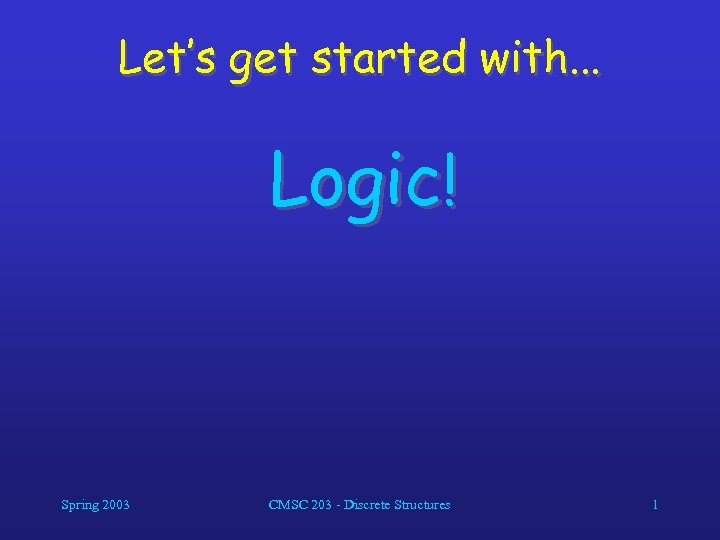Let’s get started with. . . Logic! Spring 2003 CMSC 203 - Discrete Structures 1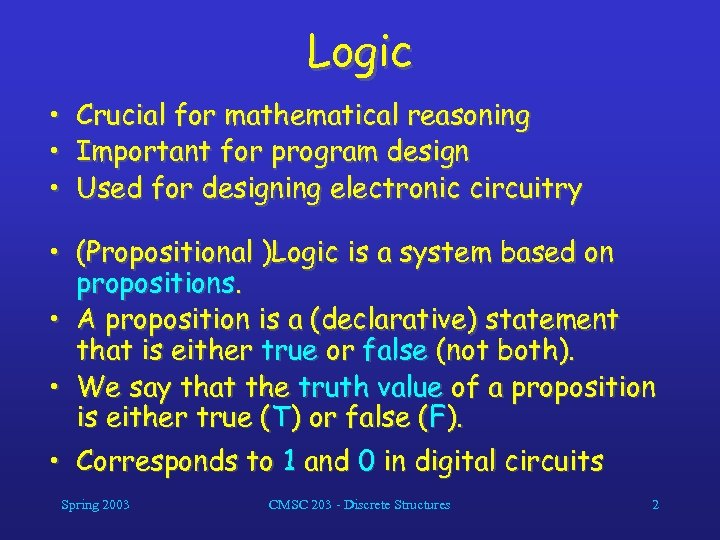Logic • • • Crucial for mathematical reasoning Important for program design Used for designing electronic circuitry • (Propositional )Logic is a system based on propositions. • A proposition is a (declarative) statement that is either true or false (not both). • We say that the truth value of a proposition is either true (T) or false (F). • Corresponds to 1 and 0 in digital circuits Spring 2003 CMSC 203 - Discrete Structures 2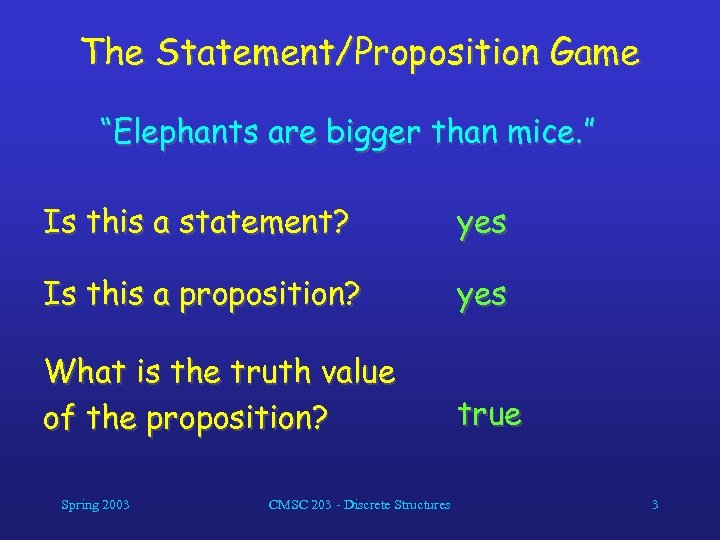The Statement/Proposition Game “Elephants are bigger than mice. ” Is this a statement? yes Is this a proposition? yes What is the truth value of the proposition? true Spring 2003 CMSC 203 - Discrete Structures 3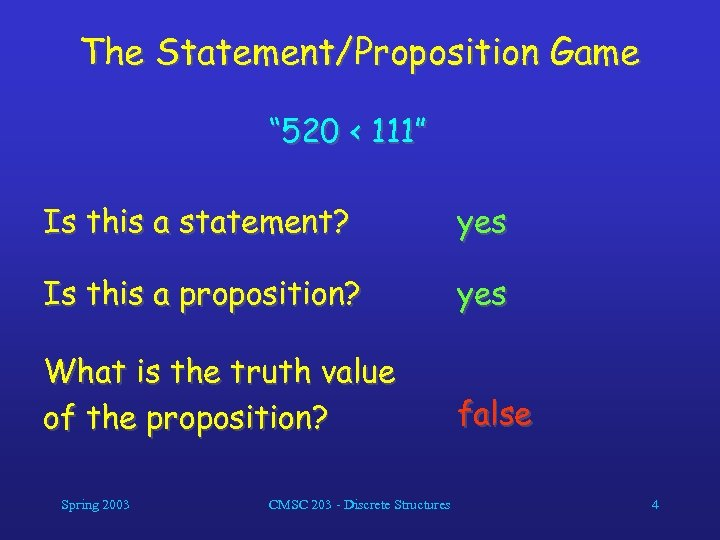The Statement/Proposition Game “ 520 < 111” Is this a statement? yes Is this a proposition? yes What is the truth value of the proposition? false Spring 2003 CMSC 203 - Discrete Structures 4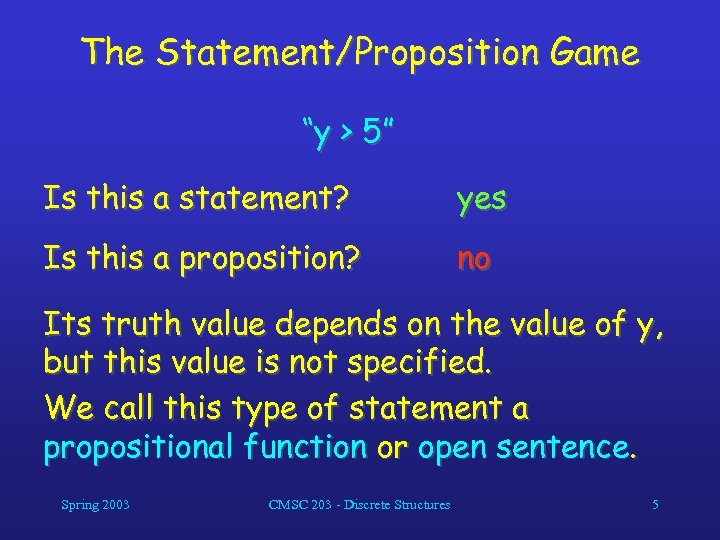The Statement/Proposition Game “y > 5” Is this a statement? yes Is this a proposition? no Its truth value depends on the value of y, but this value is not specified. We call this type of statement a propositional function or open sentence. Spring 2003 CMSC 203 - Discrete Structures 5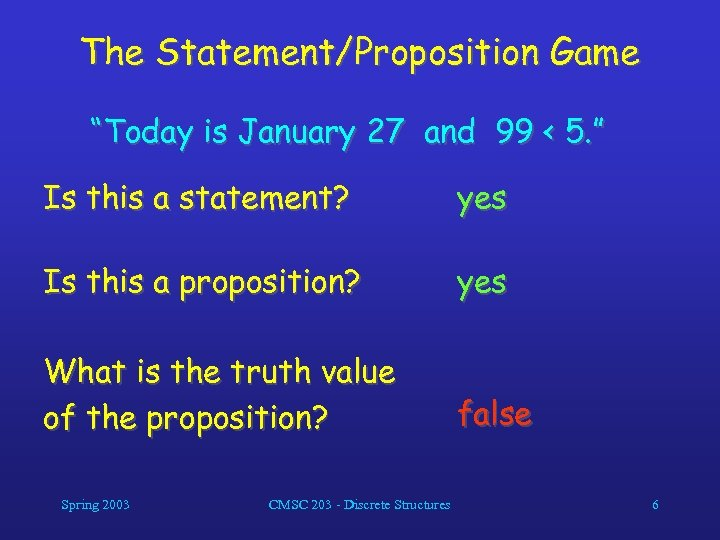The Statement/Proposition Game “Today is January 27 and 99 < 5. ” Is this a statement? yes Is this a proposition? yes What is the truth value of the proposition? false Spring 2003 CMSC 203 - Discrete Structures 6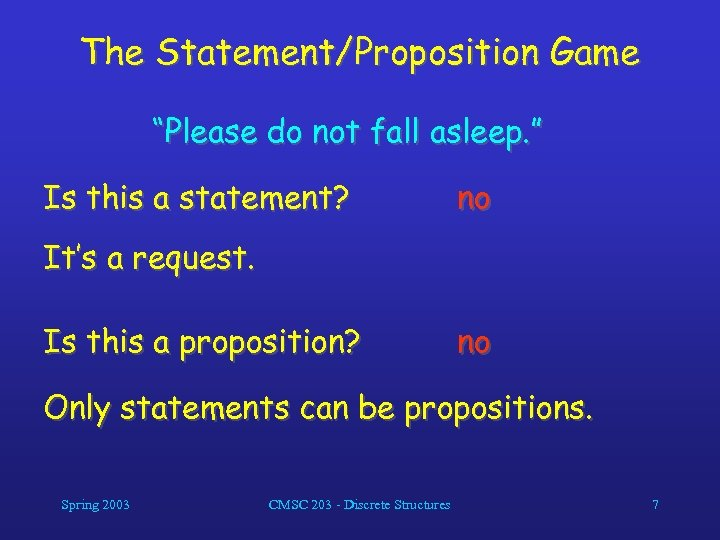The Statement/Proposition Game “Please do not fall asleep. ” Is this a statement? no It’s a request. Is this a proposition? no Only statements can be propositions. Spring 2003 CMSC 203 - Discrete Structures 7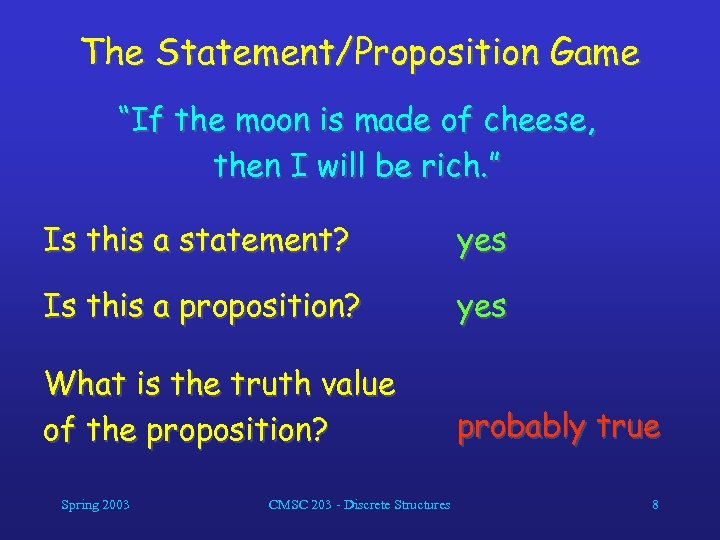The Statement/Proposition Game “If the moon is made of cheese, then I will be rich. ” Is this a statement? yes Is this a proposition? yes What is the truth value of the proposition? probably true Spring 2003 CMSC 203 - Discrete Structures 8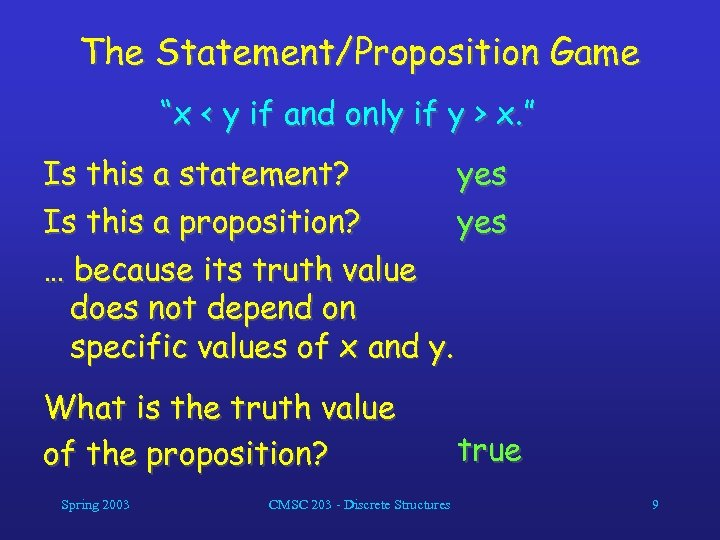The Statement/Proposition Game “x < y if and only if y > x. ” Is this a statement? yes Is this a proposition? yes … because its truth value does not depend on specific values of x and y. What is the truth value of the proposition? Spring 2003 CMSC 203 - Discrete Structures true 9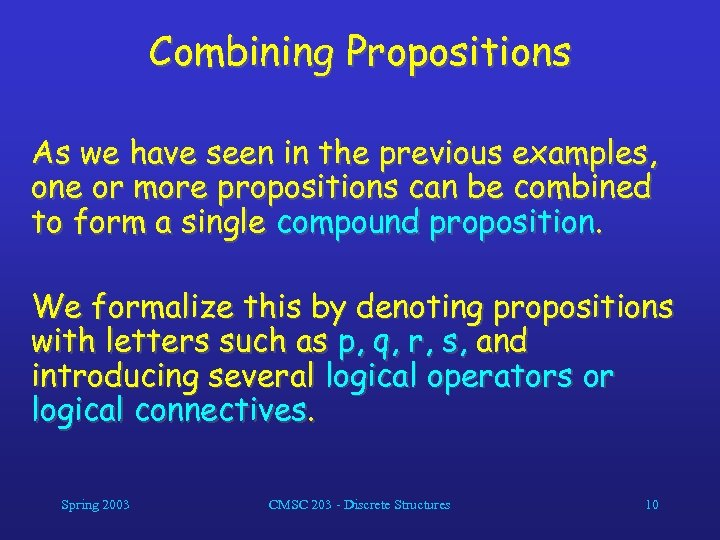Combining Propositions As we have seen in the previous examples, one or more propositions can be combined to form a single compound proposition. We formalize this by denoting propositions with letters such as p, q, r, s, and introducing several logical operators or logical connectives. Spring 2003 CMSC 203 - Discrete Structures 10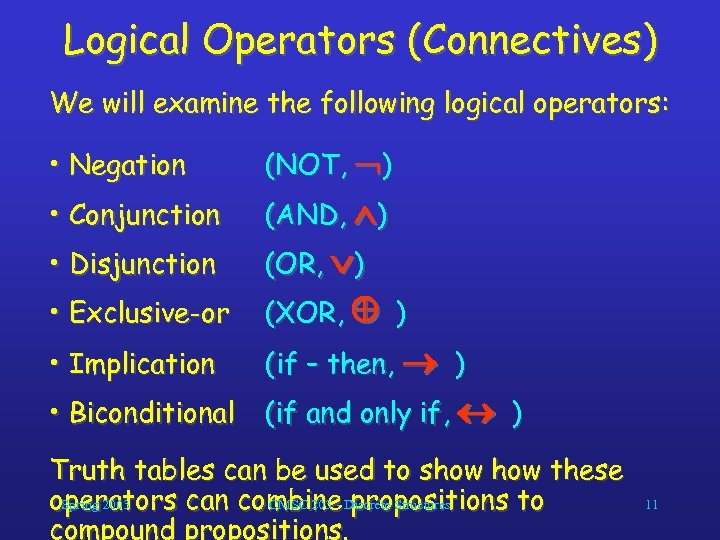Logical Operators (Connectives) We will examine the following logical operators: • Negation (NOT, ) • Conjunction (AND, ) • Disjunction (OR, ) • Exclusive-or (XOR, ) • Implication (if – then, ) • Biconditional (if and only if, ) Truth tables can be used to show these Spring 2003 CMSC 203 - propositions to operators can combine Discrete Structures compound propositions. 11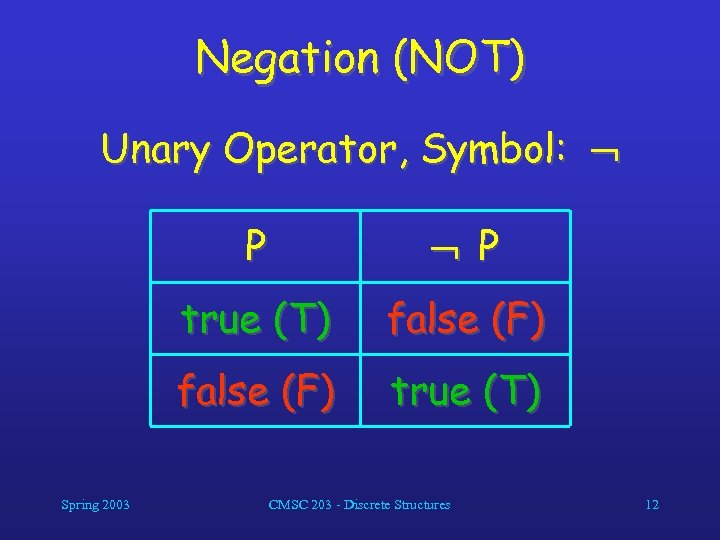Negation (NOT) Unary Operator, Symbol: P true (T) false (F) Spring 2003 P true (T) CMSC 203 - Discrete Structures 12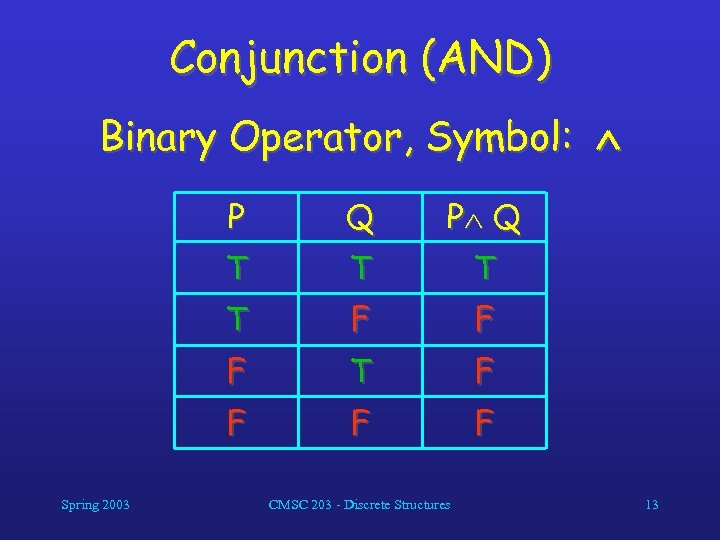Conjunction (AND) Binary Operator, Symbol: P P Q T T F F F T F F Spring 2003 Q F F CMSC 203 - Discrete Structures 13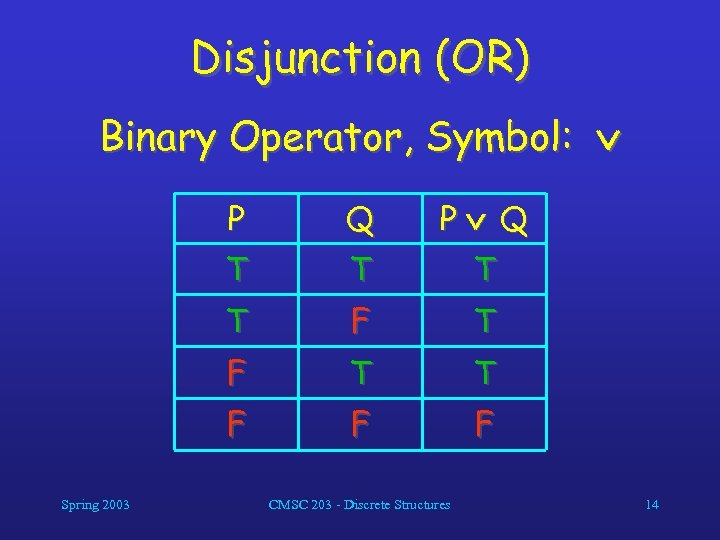Disjunction (OR) Binary Operator, Symbol: P P Q T T F Spring 2003 Q F F CMSC 203 - Discrete Structures 14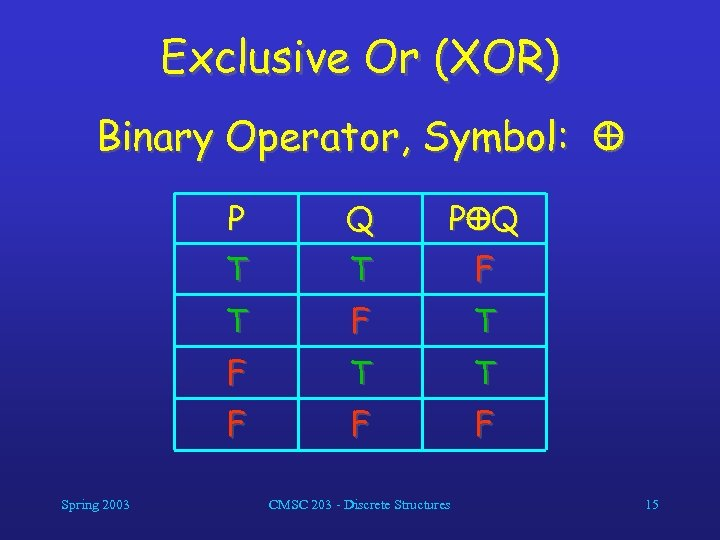Exclusive Or (XOR) Binary Operator, Symbol: P P Q T T F T F T T F Spring 2003 Q F F CMSC 203 - Discrete Structures 15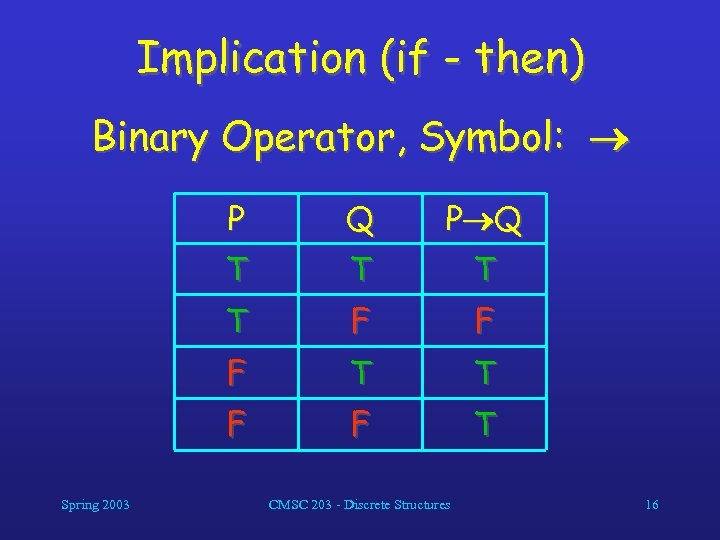Implication (if - then) Binary Operator, Symbol: P P Q T T F F F T T F Spring 2003 Q F T CMSC 203 - Discrete Structures 16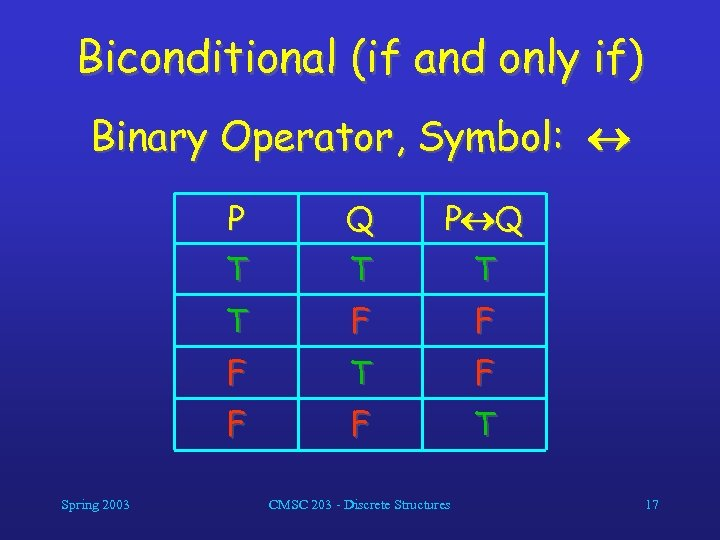Biconditional (if and only if) Binary Operator, Symbol: P P Q T T F F F T F F Spring 2003 Q F T CMSC 203 - Discrete Structures 17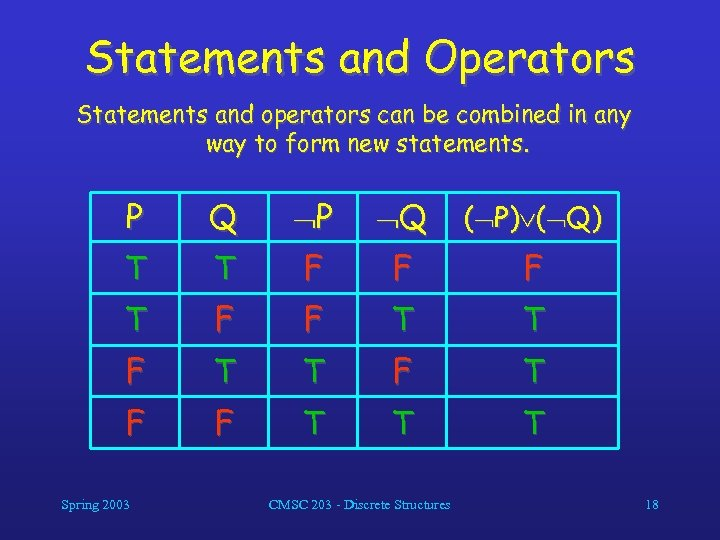Statements and Operators Statements and operators can be combined in any way to form new statements. P Q P Q ( P) ( Q) T T F F F T T F T F F T T T Spring 2003 CMSC 203 - Discrete Structures 18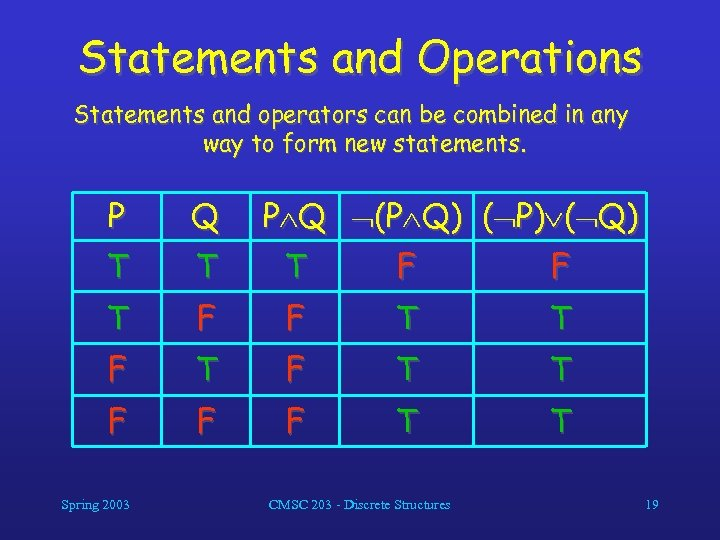Statements and Operations Statements and operators can be combined in any way to form new statements. P Q T T T F F T T F F F T T Spring 2003 P Q (P Q) ( P) ( Q) CMSC 203 - Discrete Structures 19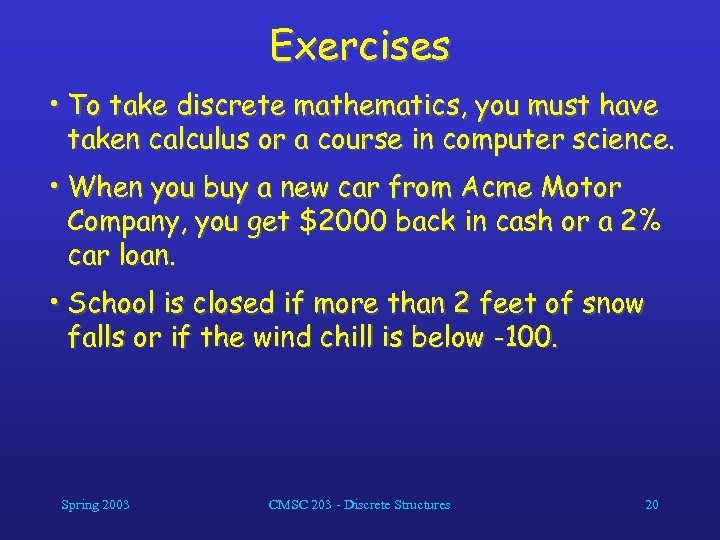Exercises • To take discrete mathematics, you must have taken calculus or a course in computer science. • When you buy a new car from Acme Motor Company, you get \$2000 back in cash or a 2% car loan. • School is closed if more than 2 feet of snow falls or if the wind chill is below -100. Spring 2003 CMSC 203 - Discrete Structures 20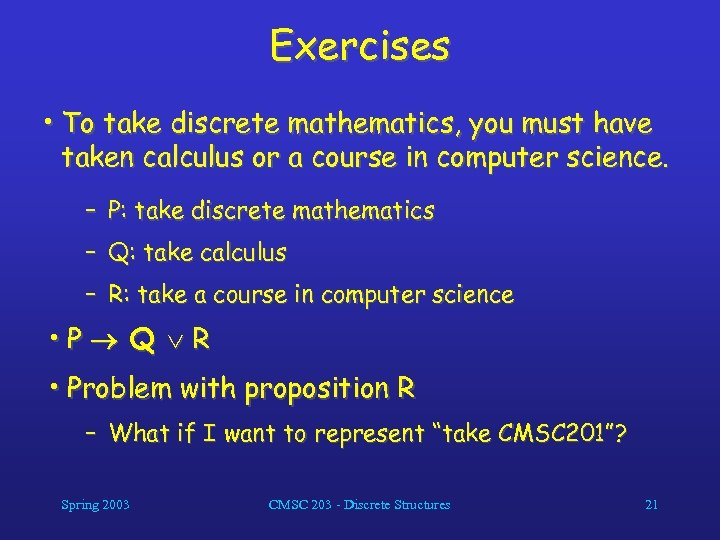Exercises • To take discrete mathematics, you must have taken calculus or a course in computer science. – P: take discrete mathematics – Q: take calculus – R: take a course in computer science • P Q R • Problem with proposition R – What if I want to represent “take CMSC 201”? Spring 2003 CMSC 203 - Discrete Structures 21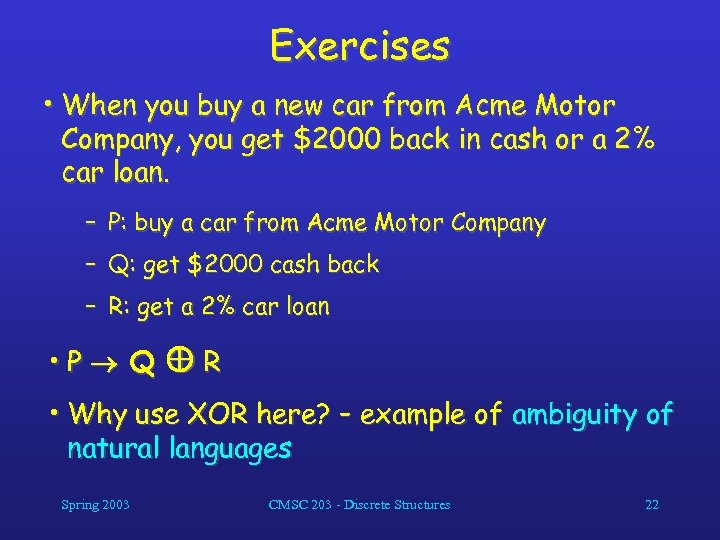Exercises • When you buy a new car from Acme Motor Company, you get \$2000 back in cash or a 2% car loan. – P: buy a car from Acme Motor Company – Q: get \$2000 cash back – R: get a 2% car loan • P Q R • Why use XOR here? – example of ambiguity of natural languages Spring 2003 CMSC 203 - Discrete Structures 22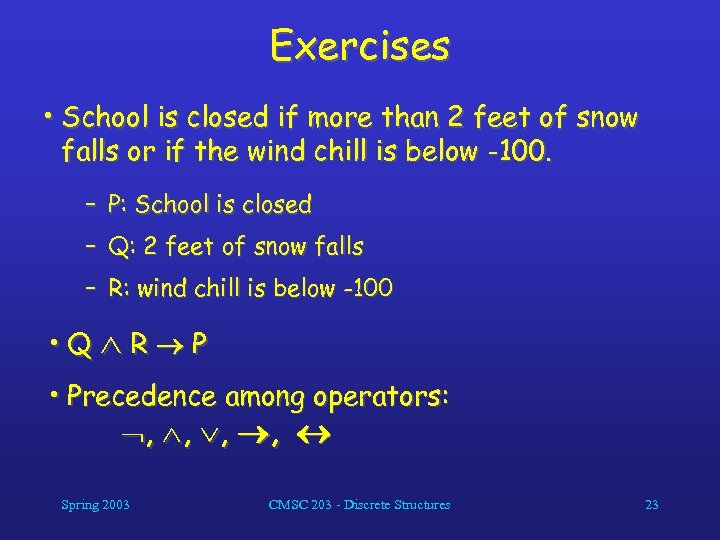Exercises • School is closed if more than 2 feet of snow falls or if the wind chill is below -100. – P: School is closed – Q: 2 feet of snow falls – R: wind chill is below -100 • Q R P • Precedence among operators: , , Spring 2003 CMSC 203 - Discrete Structures 23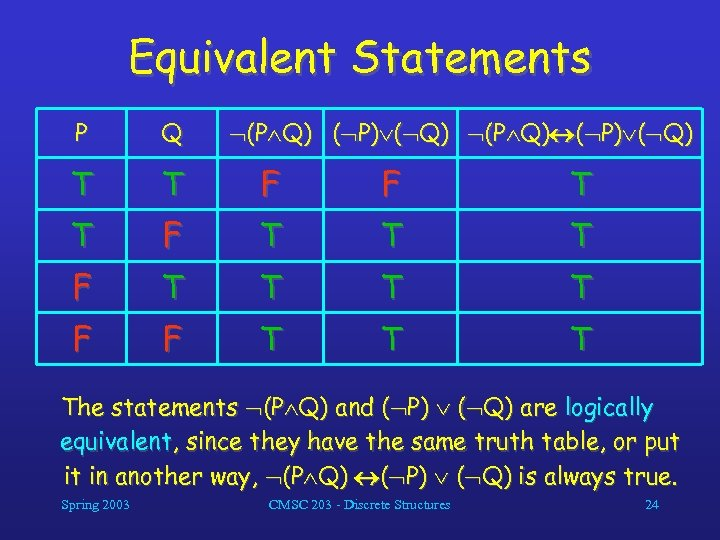Equivalent Statements P Q (P Q) ( P) ( Q) T T F F T T T F F T The statements (P Q) and ( P) ( Q) are logically equivalent, since they have the same truth table, or put it in another way, (P Q) ( P) ( Q) is always true. Spring 2003 CMSC 203 - Discrete Structures 24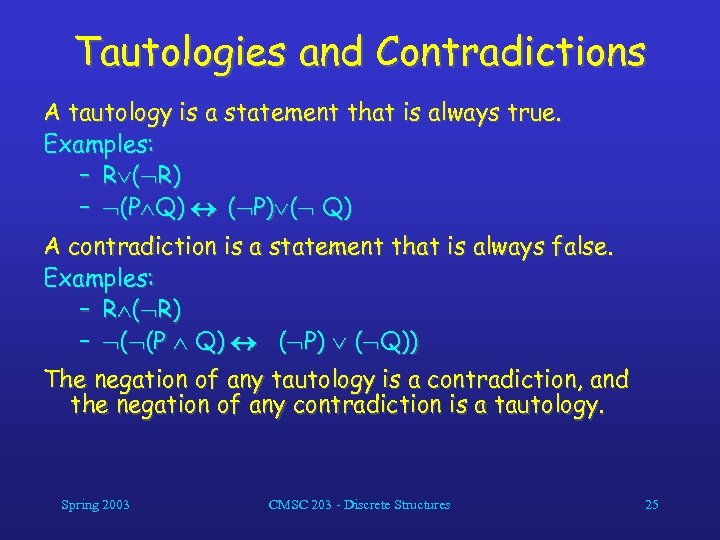Tautologies and Contradictions A tautology is a statement that is always true. Examples: – R ( R) – (P Q) ( P) ( Q) A contradiction is a statement that is always false. Examples: – R ( R) – ( (P Q) ( P) ( Q)) The negation of any tautology is a contradiction, and the negation of any contradiction is a tautology. Spring 2003 CMSC 203 - Discrete Structures 25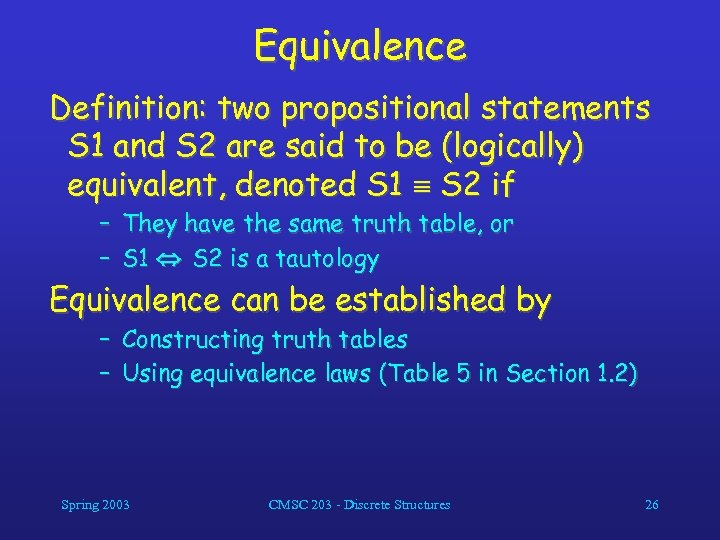Equivalence Definition: two propositional statements S 1 and S 2 are said to be (logically) equivalent, denoted S 1 S 2 if – They have the same truth table, or – S 1 S 2 is a tautology Equivalence can be established by – Constructing truth tables – Using equivalence laws (Table 5 in Section 1. 2) Spring 2003 CMSC 203 - Discrete Structures 26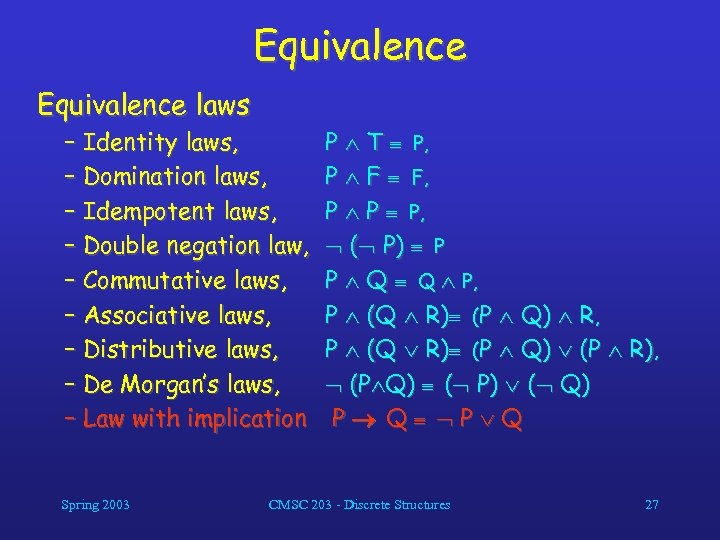Equivalence laws – Identity laws, – Domination laws, – Idempotent laws, – Double negation law, – Commutative laws, – Associative laws, – Distributive laws, – De Morgan’s laws, – Law with implication Spring 2003 P T P, P F F, P P P, ( P ) P P Q Q P, P (Q R) (P Q) R, P (Q R) (P Q) (P R), (P Q) ( P) ( Q) P Q CMSC 203 - Discrete Structures 27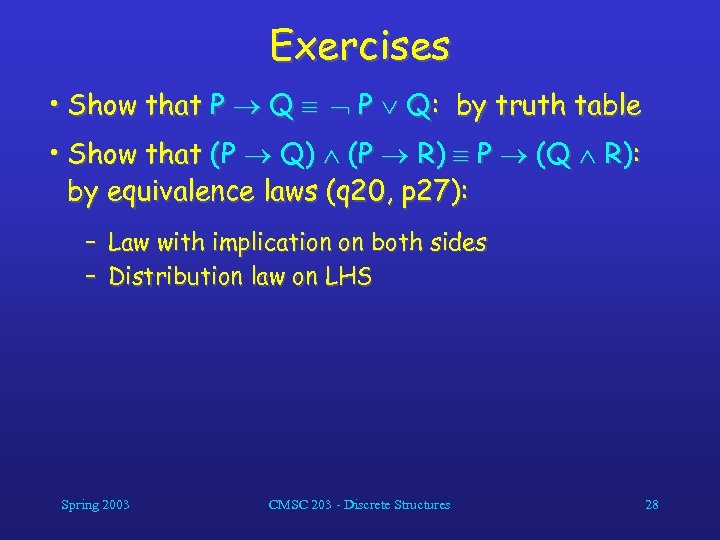Exercises • Show that P Q: by truth table • Show that (P Q) (P R) P (Q R): by equivalence laws (q 20, p 27): – Law with implication on both sides – Distribution law on LHS Spring 2003 CMSC 203 - Discrete Structures 28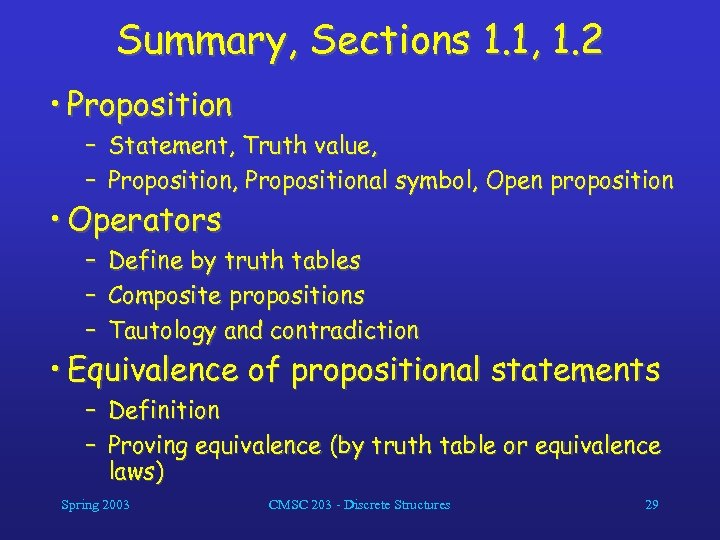Summary, Sections 1. 1, 1. 2 • Proposition – Statement, Truth value, – Proposition, Propositional symbol, Open proposition • Operators – – – Define by truth tables Composite propositions Tautology and contradiction • Equivalence of propositional statements – Definition – Proving equivalence (by truth table or equivalence laws) Spring 2003 CMSC 203 - Discrete Structures 29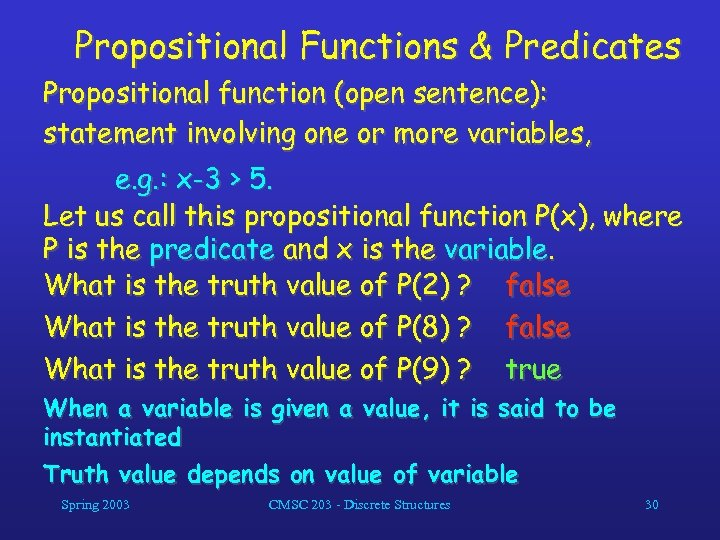Propositional Functions & Predicates Propositional function (open sentence): statement involving one or more variables, e. g. : x-3 > 5. Let us call this propositional function P(x), where P is the predicate and x is the variable. What is the truth value of P(2) ? false What is the truth value of P(8) ? false What is the truth value of P(9) ? true When a variable is given a value, it is said to be instantiated Truth value depends on value of variable Spring 2003 CMSC 203 - Discrete Structures 30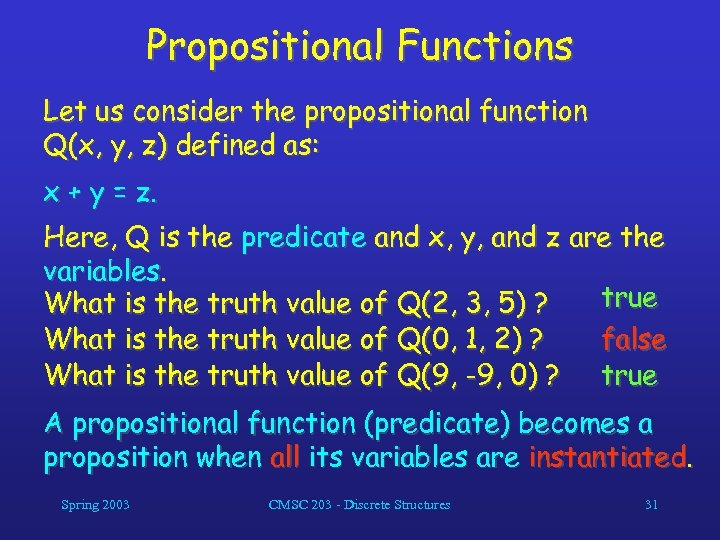Propositional Functions Let us consider the propositional function Q(x, y, z) defined as: x + y = z. Here, Q is the predicate and x, y, and z are the variables. true What is the truth value of Q(2, 3, 5) ? What is the truth value of Q(0, 1, 2) ? false What is the truth value of Q(9, -9, 0) ? true A propositional function (predicate) becomes a proposition when all its variables are instantiated. Spring 2003 CMSC 203 - Discrete Structures 31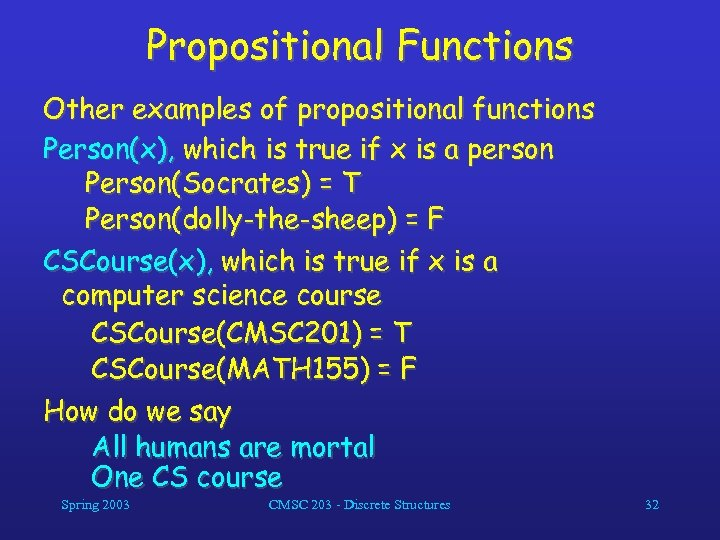Propositional Functions Other examples of propositional functions Person(x), which is true if x is a person Person(Socrates) = T Person(dolly-the-sheep) = F CSCourse(x), which is true if x is a computer science course CSCourse(CMSC 201) = T CSCourse(MATH 155) = F How do we say All humans are mortal One CS course Spring 2003 CMSC 203 - Discrete Structures 32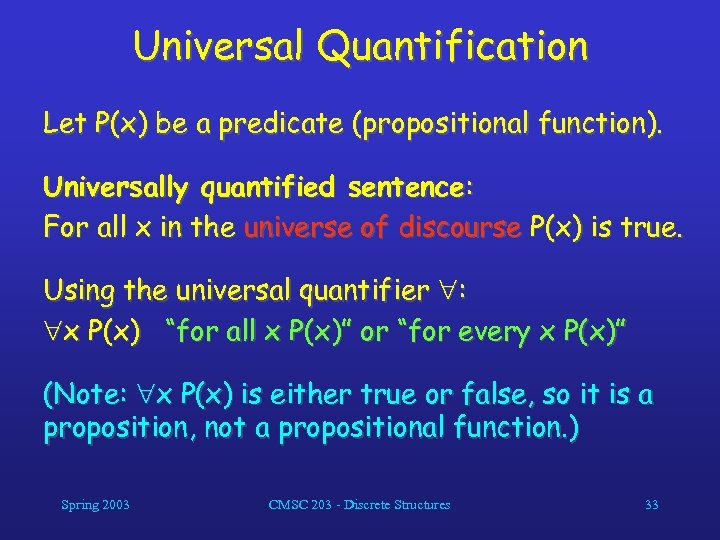Universal Quantification Let P(x) be a predicate (propositional function). Universally quantified sentence: For all x in the universe of discourse P(x) is true. Using the universal quantifier : x P(x) “for all x P(x)” or “for every x P(x)” (Note: x P(x) is either true or false, so it is a proposition, not a propositional function. ) Spring 2003 CMSC 203 - Discrete Structures 33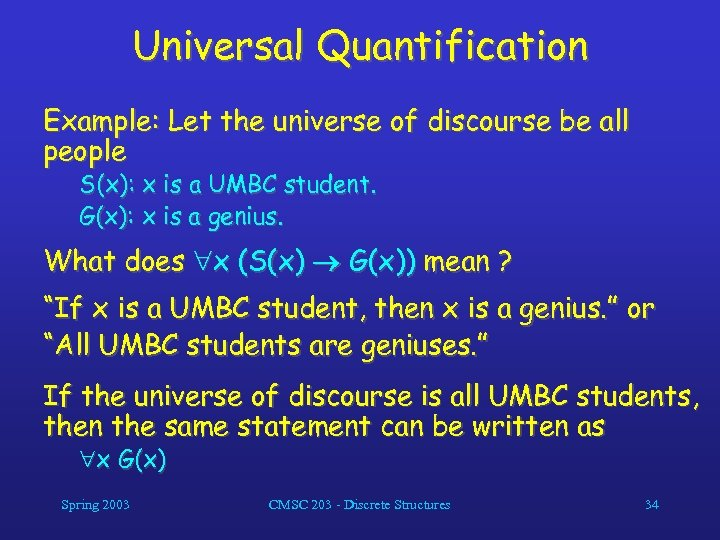Universal Quantification Example: Let the universe of discourse be all people S(x): x is a UMBC student. G(x): x is a genius. What does x (S(x) G(x)) mean ? “If x is a UMBC student, then x is a genius. ” or “All UMBC students are geniuses. ” If the universe of discourse is all UMBC students, then the same statement can be written as x G(x) Spring 2003 CMSC 203 - Discrete Structures 34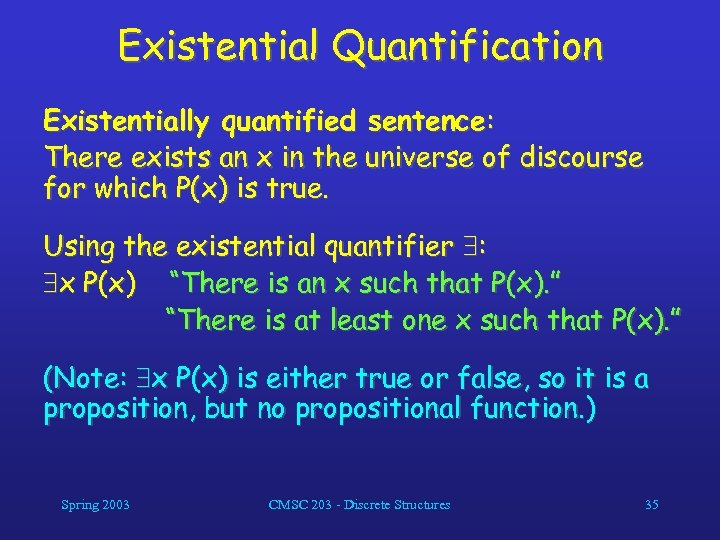Existential Quantification Existentially quantified sentence: There exists an x in the universe of discourse for which P(x) is true. Using the existential quantifier : x P(x) “There is an x such that P(x). ” “There is at least one x such that P(x). ” (Note: x P(x) is either true or false, so it is a proposition, but no propositional function. ) Spring 2003 CMSC 203 - Discrete Structures 35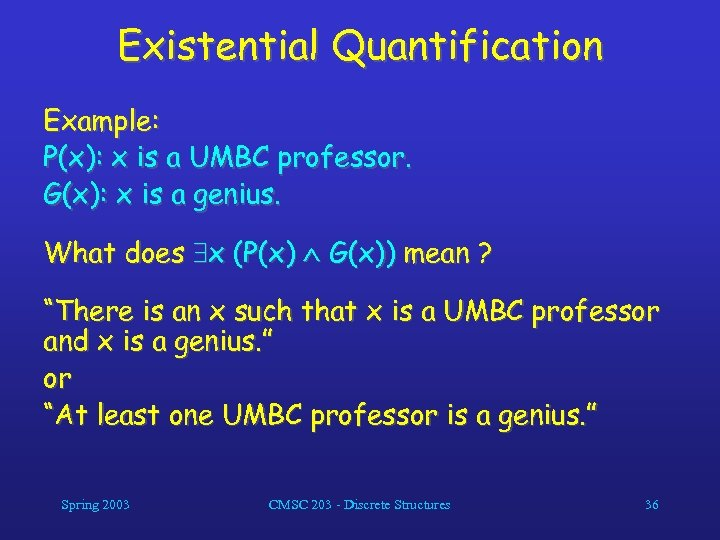Existential Quantification Example: P(x): x is a UMBC professor. G(x): x is a genius. What does x (P(x) G(x)) mean ? “There is an x such that x is a UMBC professor and x is a genius. ” or “At least one UMBC professor is a genius. ” Spring 2003 CMSC 203 - Discrete Structures 36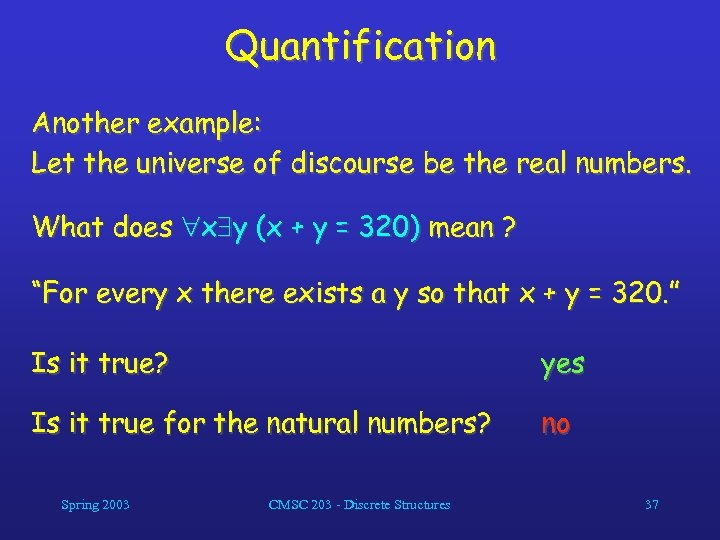Quantification Another example: Let the universe of discourse be the real numbers. What does x y (x + y = 320) mean ? “For every x there exists a y so that x + y = 320. ” Is it true? yes Is it true for the natural numbers? no Spring 2003 CMSC 203 - Discrete Structures 37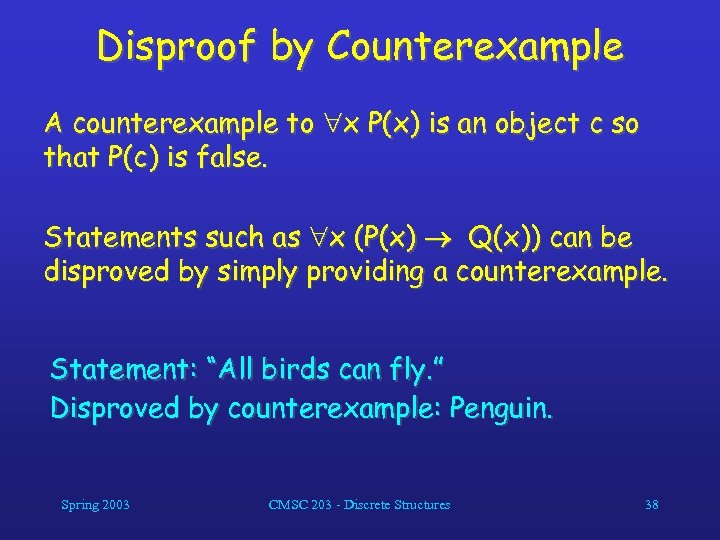Disproof by Counterexample A counterexample to x P(x) is an object c so that P(c) is false. Statements such as x (P(x) Q(x)) can be disproved by simply providing a counterexample. Statement: “All birds can fly. ” Disproved by counterexample: Penguin. Spring 2003 CMSC 203 - Discrete Structures 38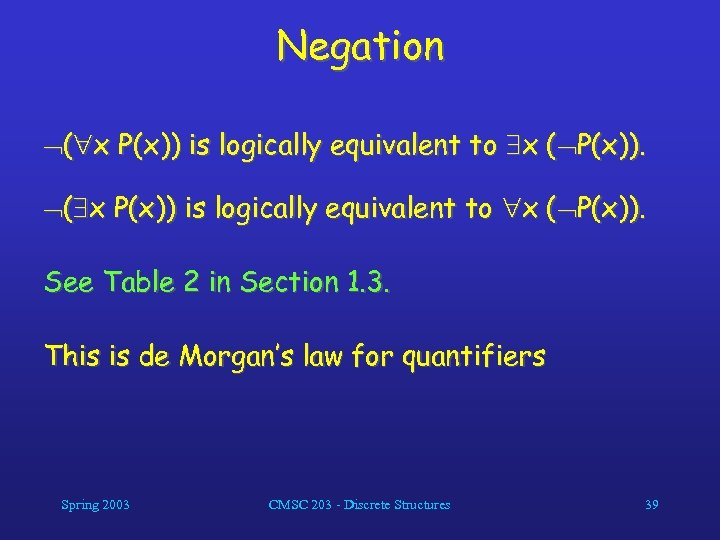Negation ( x P(x)) is logically equivalent to x ( P(x)). See Table 2 in Section 1. 3. This is de Morgan’s law for quantifiers Spring 2003 CMSC 203 - Discrete Structures 39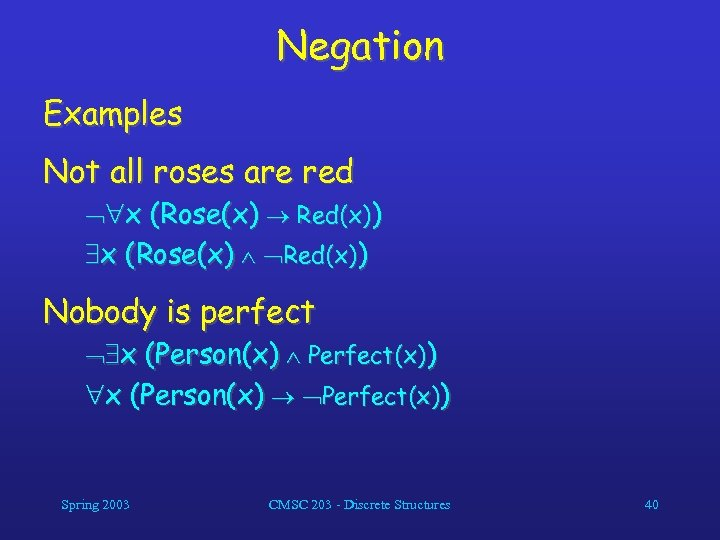Negation Examples Not all roses are red x (Rose(x) Red(x)) Nobody is perfect x (Person(x) Perfect(x)) Spring 2003 CMSC 203 - Discrete Structures 40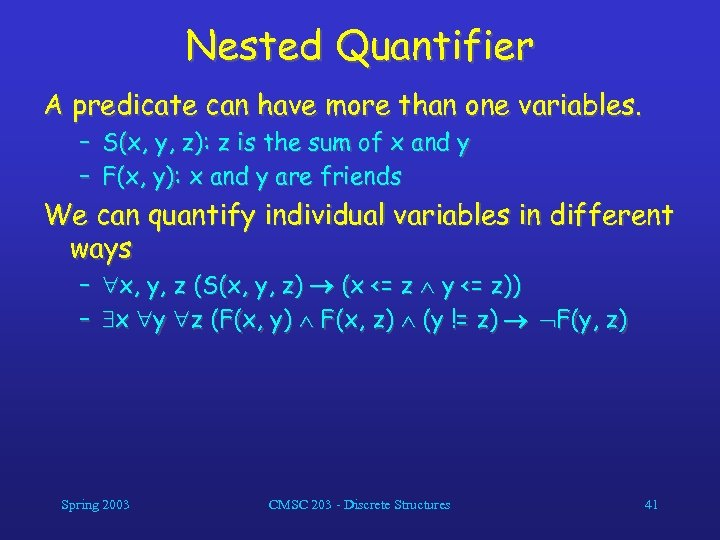Nested Quantifier A predicate can have more than one variables. – S(x, y, z): z is the sum of x and y – F(x, y): x and y are friends We can quantify individual variables in different ways – x, y, z (S(x, y, z) (x <= z y <= z)) – x y z (F(x, y) F(x, z) (y != z) F(y, z) Spring 2003 CMSC 203 - Discrete Structures 41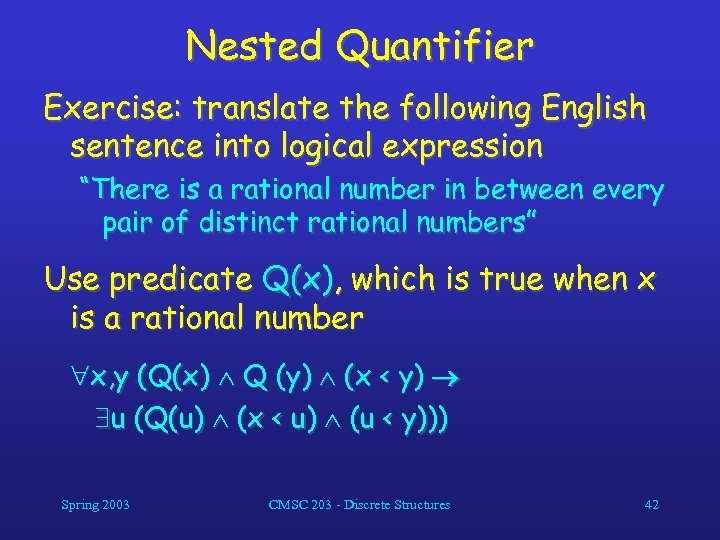Nested Quantifier Exercise: translate the following English sentence into logical expression “There is a rational number in between every pair of distinct rational numbers” Use predicate Q(x), which is true when x is a rational number x, y (Q(x) Q (y) (x < y) u (Q(u) (x < u) (u < y))) Spring 2003 CMSC 203 - Discrete Structures 42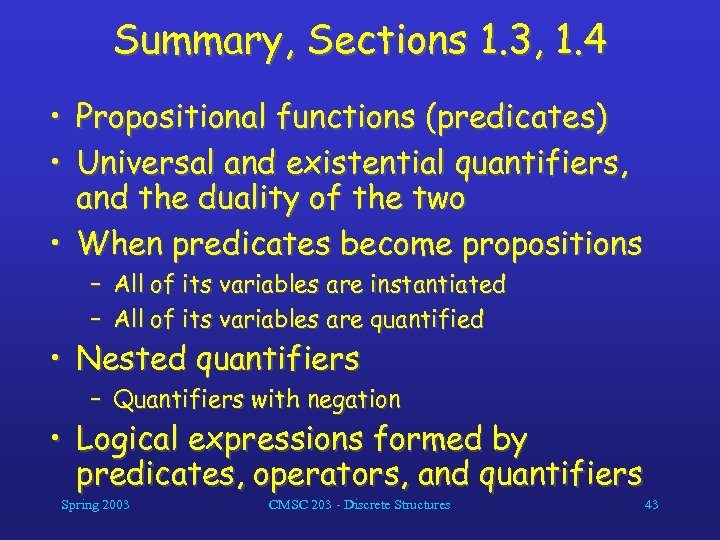Summary, Sections 1. 3, 1. 4 • Propositional functions (predicates) • Universal and existential quantifiers, and the duality of the two • When predicates become propositions – All of its variables are instantiated – All of its variables are quantified • Nested quantifiers – Quantifiers with negation • Logical expressions formed by predicates, operators, and quantifiers Spring 2003 CMSC 203 - Discrete Structures 43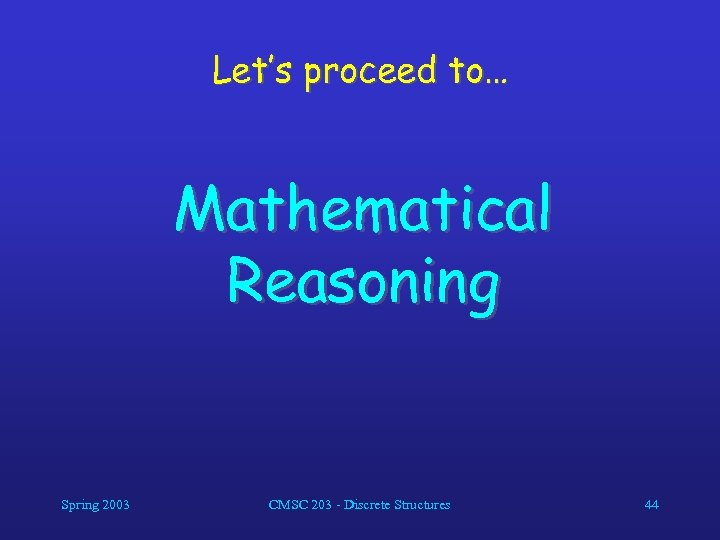Let’s proceed to… Mathematical Reasoning Spring 2003 CMSC 203 - Discrete Structures 44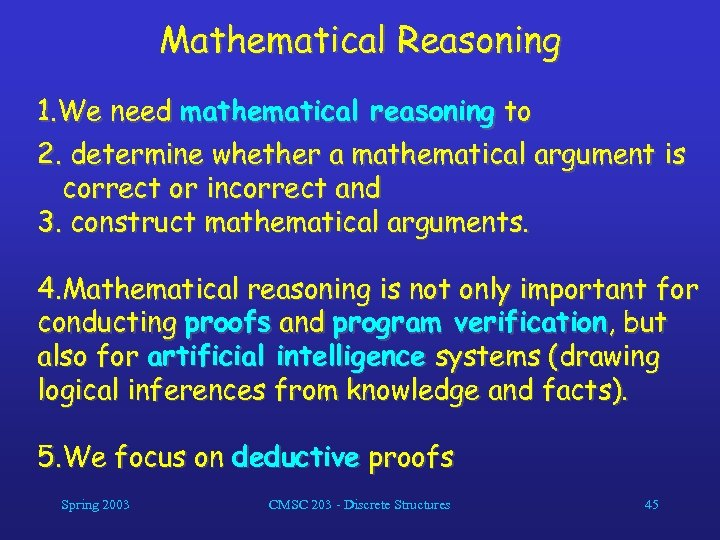Mathematical Reasoning 1. We need mathematical reasoning to 2. determine whether a mathematical argument is correct or incorrect and 3. construct mathematical arguments. 4. Mathematical reasoning is not only important for conducting proofs and program verification, but also for artificial intelligence systems (drawing logical inferences from knowledge and facts). 5. We focus on deductive proofs Spring 2003 CMSC 203 - Discrete Structures 45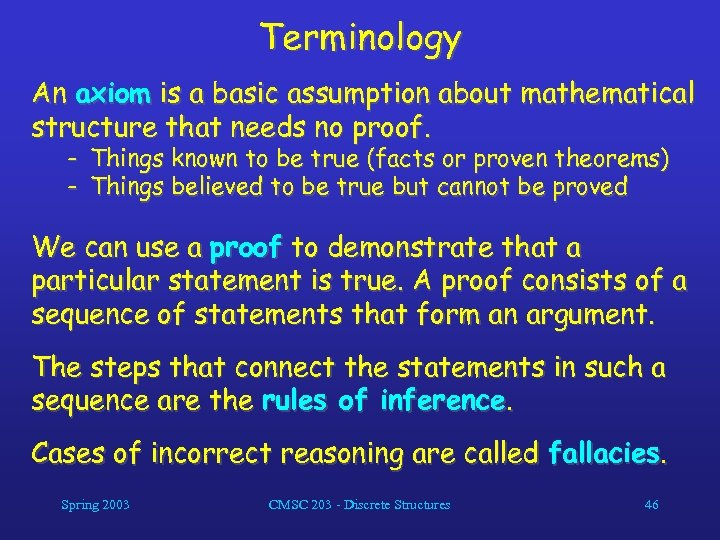Terminology An axiom is a basic assumption about mathematical structure that needs no proof. - Things known to be true (facts or proven theorems) - Things believed to be true but cannot be proved We can use a proof to demonstrate that a particular statement is true. A proof consists of a sequence of statements that form an argument. The steps that connect the statements in such a sequence are the rules of inference. Cases of incorrect reasoning are called fallacies. Spring 2003 CMSC 203 - Discrete Structures 46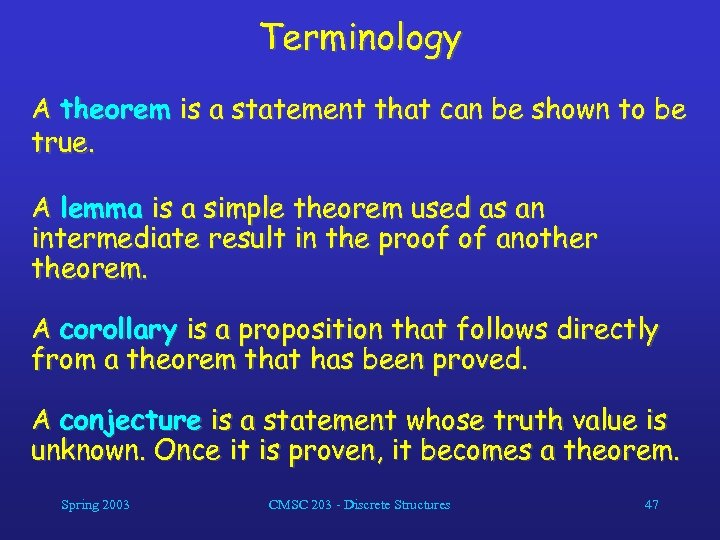Terminology A theorem is a statement that can be shown to be true. A lemma is a simple theorem used as an intermediate result in the proof of another theorem. A corollary is a proposition that follows directly from a theorem that has been proved. A conjecture is a statement whose truth value is unknown. Once it is proven, it becomes a theorem. Spring 2003 CMSC 203 - Discrete Structures 47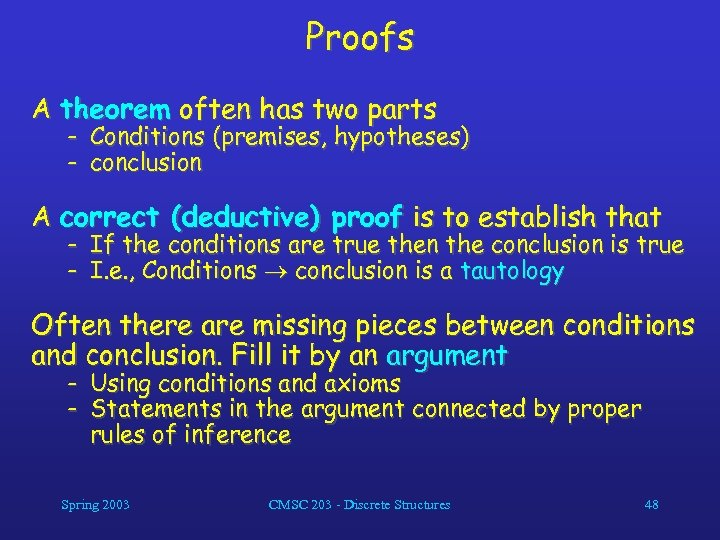Proofs A theorem often has two parts - Conditions (premises, hypotheses) - conclusion A correct (deductive) proof is to establish that - If the conditions are true then the conclusion is true - I. e. , Conditions conclusion is a tautology Often there are missing pieces between conditions and conclusion. Fill it by an argument - Using conditions and axioms - Statements in the argument connected by proper rules of inference Spring 2003 CMSC 203 - Discrete Structures 48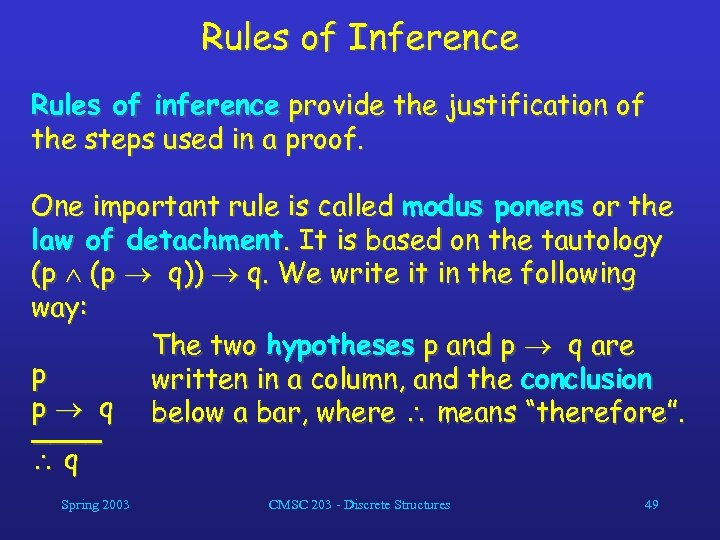Rules of Inference Rules of inference provide the justification of the steps used in a proof. One important rule is called modus ponens or the law of detachment. It is based on the tautology (p q)) q. We write it in the following way: The two hypotheses p and p q are p written in a column, and the conclusion p q below a bar, where means “therefore”. ____ q Spring 2003 CMSC 203 - Discrete Structures 49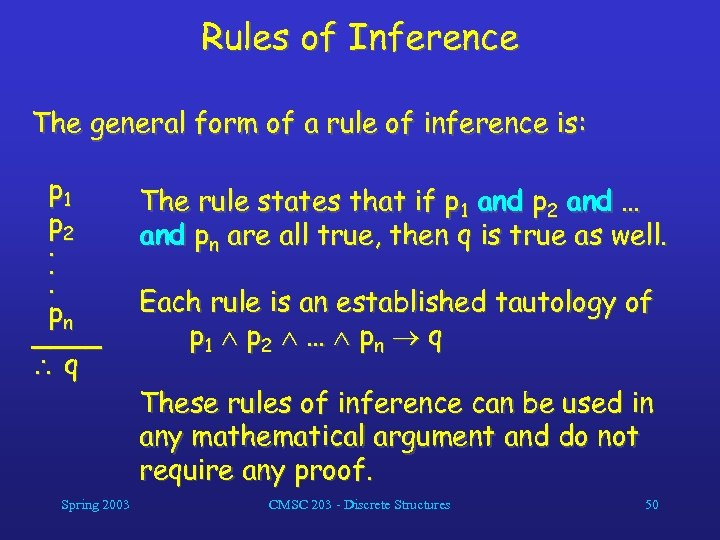Rules of Inference The general form of a rule of inference is: p 1 p 2. . . pn ____ q Spring 2003 The rule states that if p 1 and p 2 and … and pn are all true, then q is true as well. Each rule is an established tautology of p 1 p 2 … pn q These rules of inference can be used in any mathematical argument and do not require any proof. CMSC 203 - Discrete Structures 50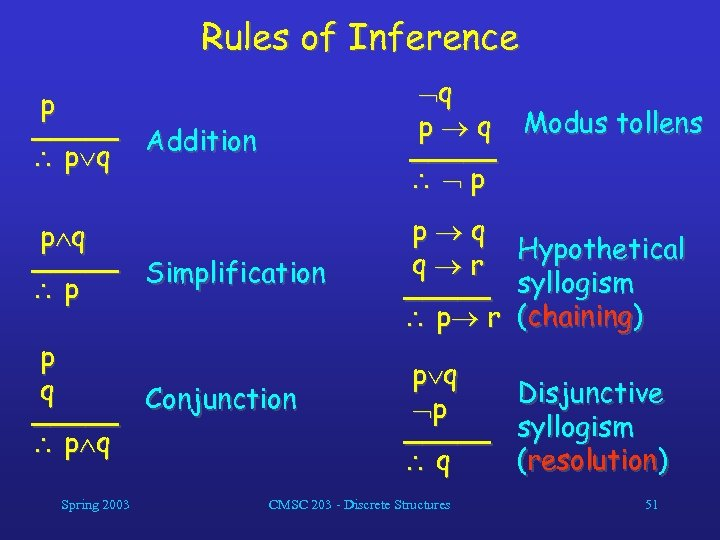Rules of Inference p _____ Addition p q q p q Modus tollens _____ p p q _____ Simplification p p q q r _____ p r Hypothetical syllogism (chaining) p q p _____ q Disjunctive syllogism (resolution) p q _____ Conjunction p q Spring 2003 CMSC 203 - Discrete Structures 51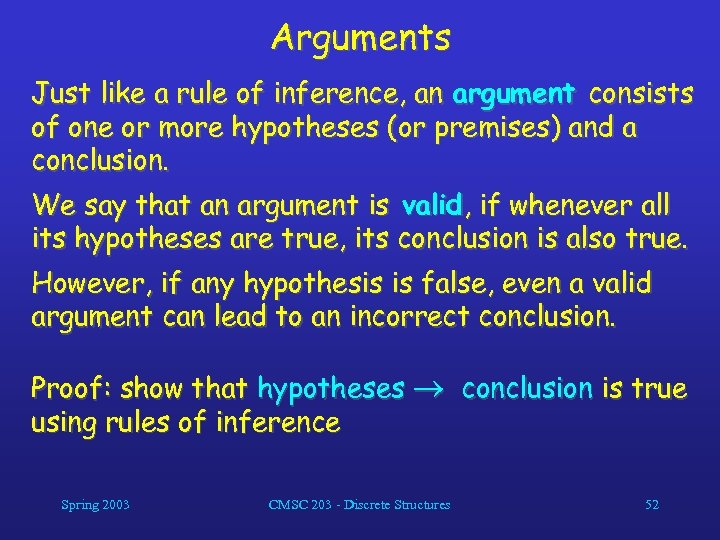Arguments Just like a rule of inference, an argument consists of one or more hypotheses (or premises) and a conclusion. We say that an argument is valid, if whenever all its hypotheses are true, its conclusion is also true. However, if any hypothesis is false, even a valid argument can lead to an incorrect conclusion. Proof: show that hypotheses conclusion is true using rules of inference Spring 2003 CMSC 203 - Discrete Structures 52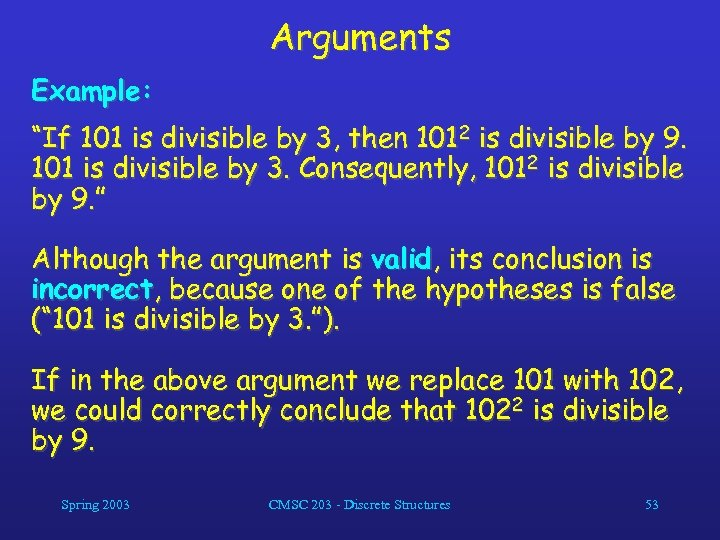Arguments Example: “If 101 is divisible by 3, then 1012 is divisible by 9. 101 is divisible by 3. Consequently, 1012 is divisible by 9. ” Although the argument is valid, its conclusion is incorrect, because one of the hypotheses is false (“ 101 is divisible by 3. ”). If in the above argument we replace 101 with 102, we could correctly conclude that 1022 is divisible by 9. Spring 2003 CMSC 203 - Discrete Structures 53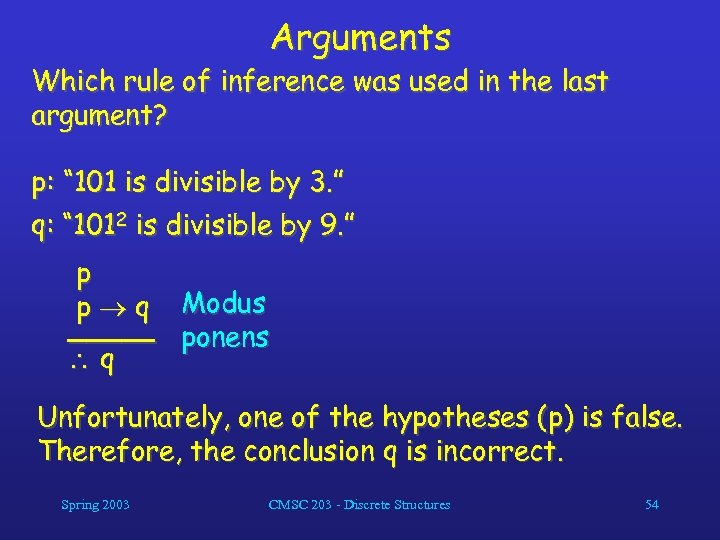Arguments Which rule of inference was used in the last argument? p: “ 101 is divisible by 3. ” q: “ 1012 is divisible by 9. ” p p q Modus _____ ponens q Unfortunately, one of the hypotheses (p) is false. Therefore, the conclusion q is incorrect. Spring 2003 CMSC 203 - Discrete Structures 54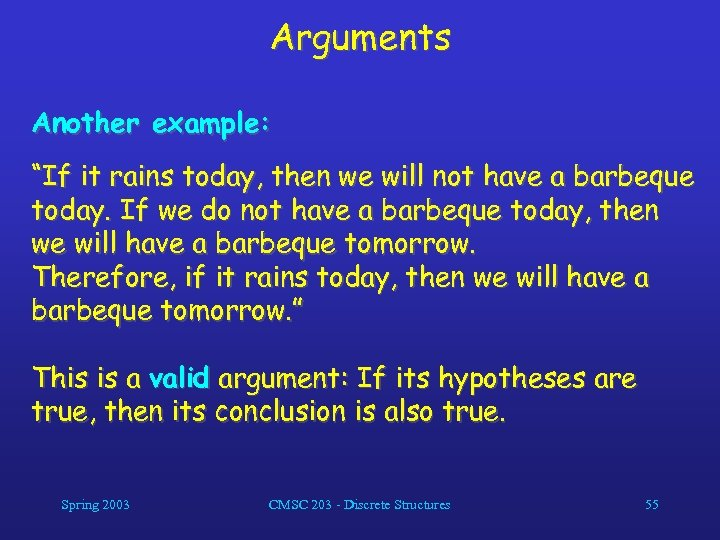Arguments Another example: “If it rains today, then we will not have a barbeque today. If we do not have a barbeque today, then we will have a barbeque tomorrow. Therefore, if it rains today, then we will have a barbeque tomorrow. ” This is a valid argument: If its hypotheses are true, then its conclusion is also true. Spring 2003 CMSC 203 - Discrete Structures 55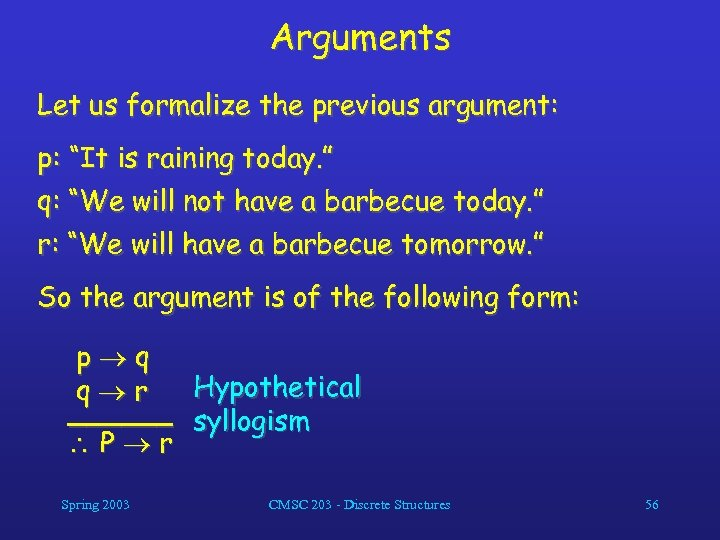Arguments Let us formalize the previous argument: p: “It is raining today. ” q: “We will not have a barbecue today. ” r: “We will have a barbecue tomorrow. ” So the argument is of the following form: p q Hypothetical q r ______ syllogism P r Spring 2003 CMSC 203 - Discrete Structures 56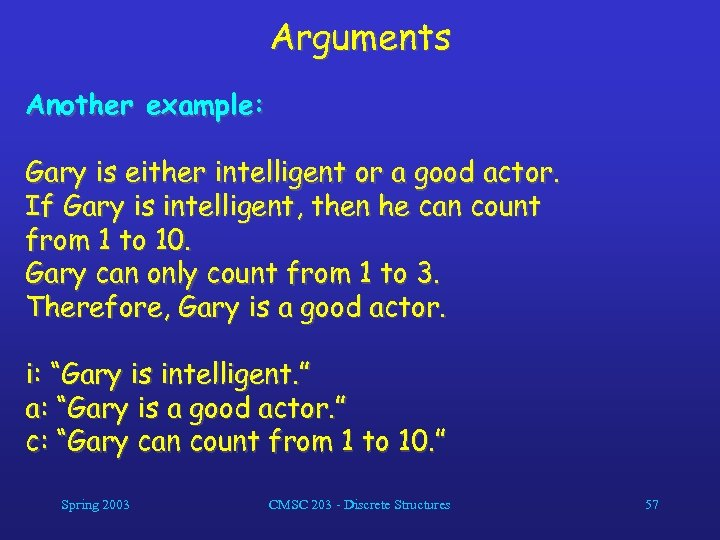Arguments Another example: Gary is either intelligent or a good actor. If Gary is intelligent, then he can count from 1 to 10. Gary can only count from 1 to 3. Therefore, Gary is a good actor. i: “Gary is intelligent. ” a: “Gary is a good actor. ” c: “Gary can count from 1 to 10. ” Spring 2003 CMSC 203 - Discrete Structures 57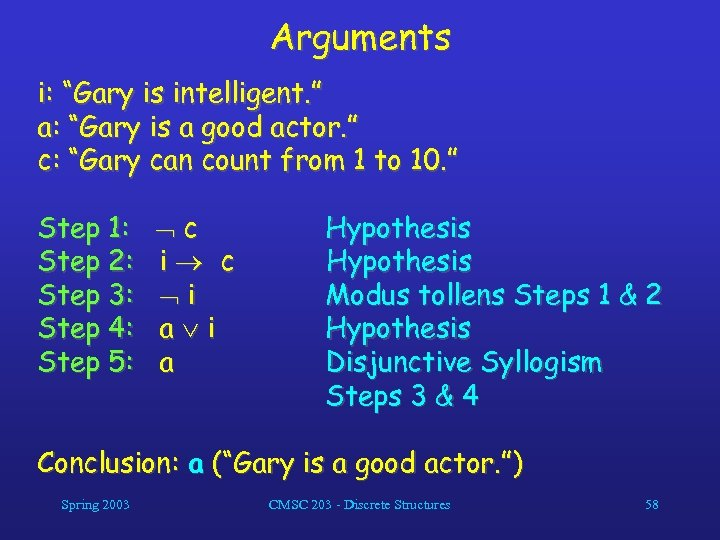Arguments i: “Gary is intelligent. ” a: “Gary is a good actor. ” c: “Gary can count from 1 to 10. ” Step 1: Step 2: Step 3: Step 4: Step 5: c i a i a Hypothesis Modus tollens Steps 1 & 2 Hypothesis Disjunctive Syllogism Steps 3 & 4 Conclusion: a (“Gary is a good actor. ”) Spring 2003 CMSC 203 - Discrete Structures 58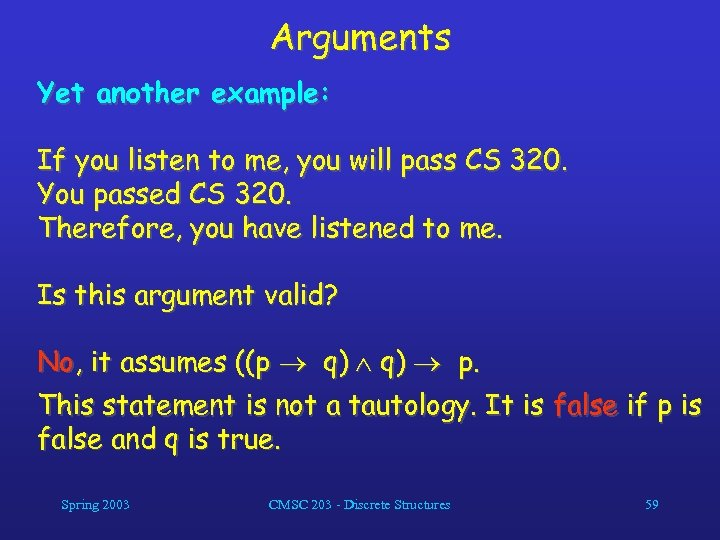Arguments Yet another example: If you listen to me, you will pass CS 320. You passed CS 320. Therefore, you have listened to me. Is this argument valid? No, it assumes ((p q) p. This statement is not a tautology. It is false if p is false and q is true. Spring 2003 CMSC 203 - Discrete Structures 59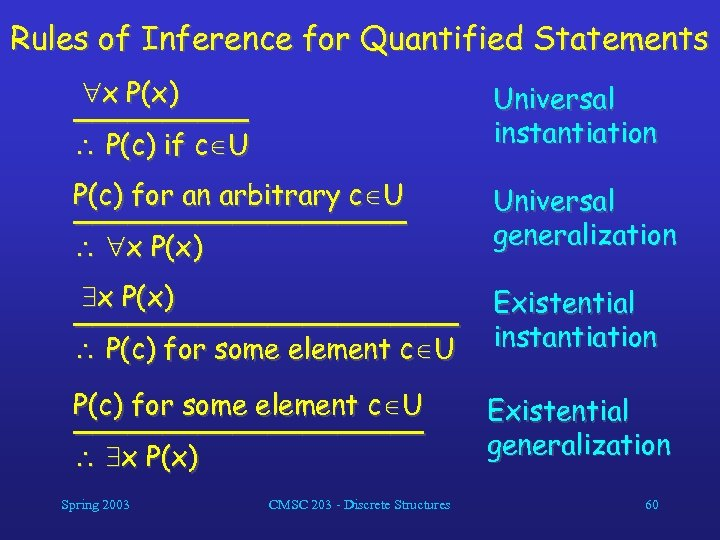Rules of Inference for Quantified Statements x P(x) _____ P(c) if c U Universal instantiation P(c) for an arbitrary c U __________ x P(x) Universal generalization x P(x) ___________ P(c) for some element c U Existential instantiation P(c) for some element c U __________ x P(x) Existential generalization Spring 2003 CMSC 203 - Discrete Structures 60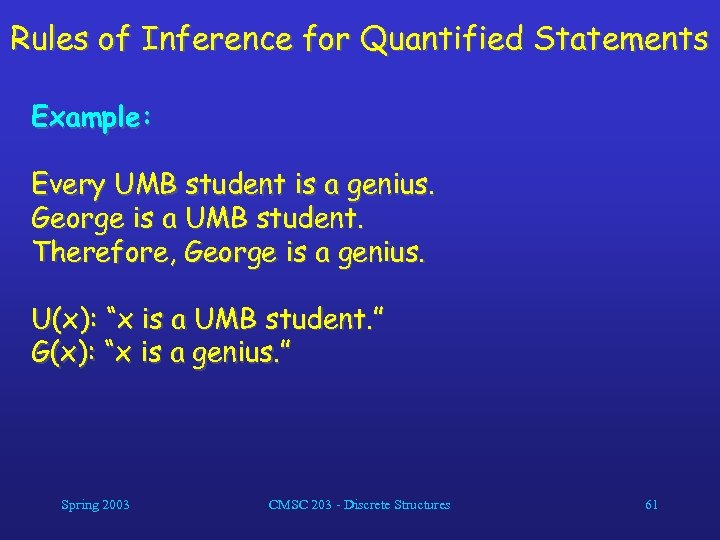Rules of Inference for Quantified Statements Example: Every UMB student is a genius. George is a UMB student. Therefore, George is a genius. U(x): “x is a UMB student. ” G(x): “x is a genius. ” Spring 2003 CMSC 203 - Discrete Structures 61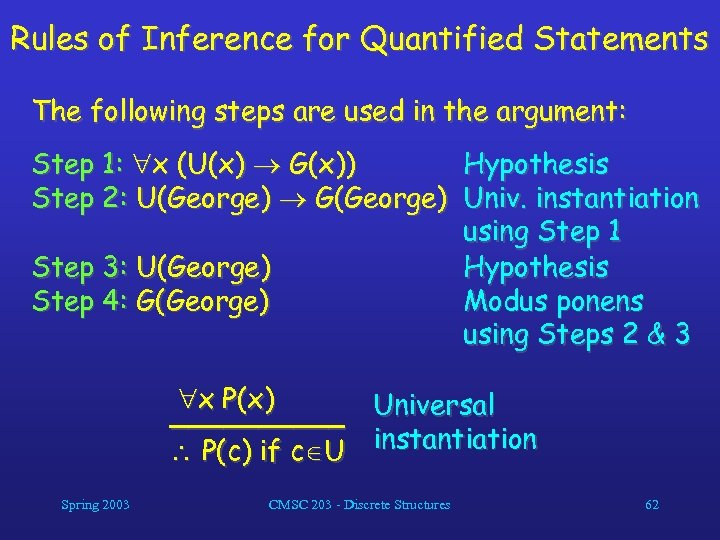Rules of Inference for Quantified Statements The following steps are used in the argument: Step 1: x (U(x) G(x)) Hypothesis Step 2: U(George) G(George) Univ. instantiation using Step 1 Step 3: U(George) Hypothesis Step 4: G(George) Modus ponens using Steps 2 & 3 x P(x) _____ Universal instantiation P(c) if c U Spring 2003 CMSC 203 - Discrete Structures 62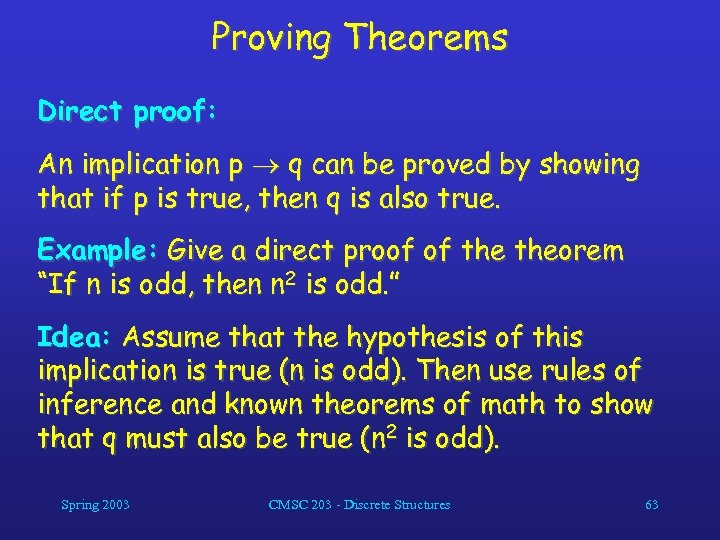Proving Theorems Direct proof: An implication p q can be proved by showing that if p is true, then q is also true. Example: Give a direct proof of theorem “If n is odd, then n 2 is odd. ” Idea: Assume that the hypothesis of this implication is true (n is odd). Then use rules of inference and known theorems of math to show that q must also be true (n 2 is odd). Spring 2003 CMSC 203 - Discrete Structures 63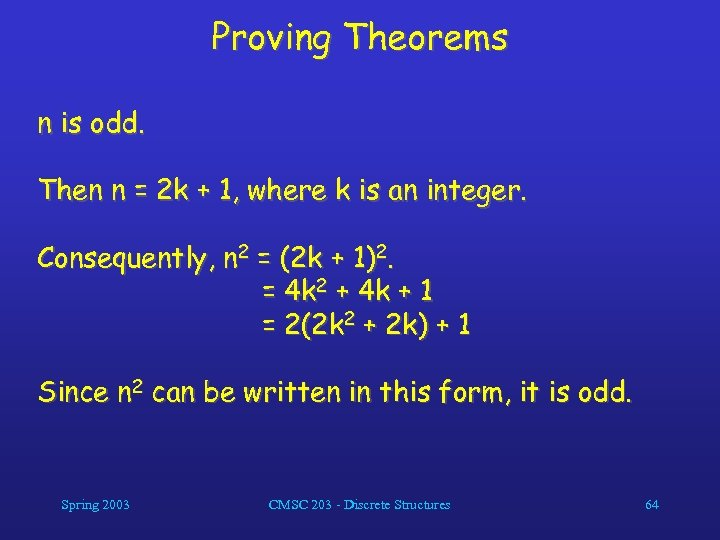Proving Theorems n is odd. Then n = 2 k + 1, where k is an integer. Consequently, n 2 = (2 k + 1)2. = 4 k 2 + 4 k + 1 = 2(2 k 2 + 2 k) + 1 Since n 2 can be written in this form, it is odd. Spring 2003 CMSC 203 - Discrete Structures 64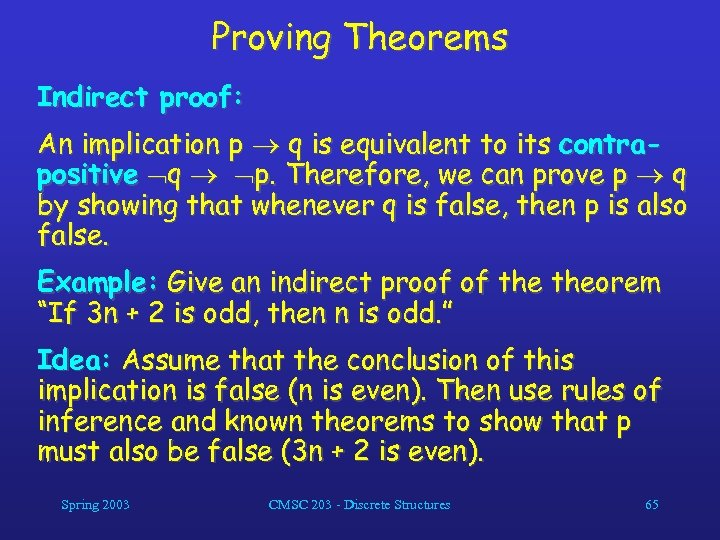Proving Theorems Indirect proof: An implication p q is equivalent to its contrapositive q p. Therefore, we can prove p q by showing that whenever q is false, then p is also false. Example: Give an indirect proof of theorem “If 3 n + 2 is odd, then n is odd. ” Idea: Assume that the conclusion of this implication is false (n is even). Then use rules of inference and known theorems to show that p must also be false (3 n + 2 is even). Spring 2003 CMSC 203 - Discrete Structures 65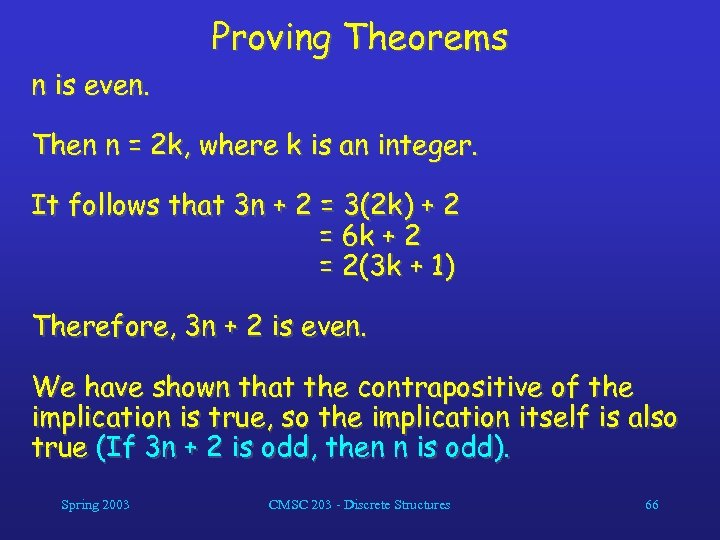Proving Theorems n is even. Then n = 2 k, where k is an integer. It follows that 3 n + 2 = 3(2 k) + 2 = 6 k + 2 = 2(3 k + 1) Therefore, 3 n + 2 is even. We have shown that the contrapositive of the implication is true, so the implication itself is also true (If 3 n + 2 is odd, then n is odd). Spring 2003 CMSC 203 - Discrete Structures 66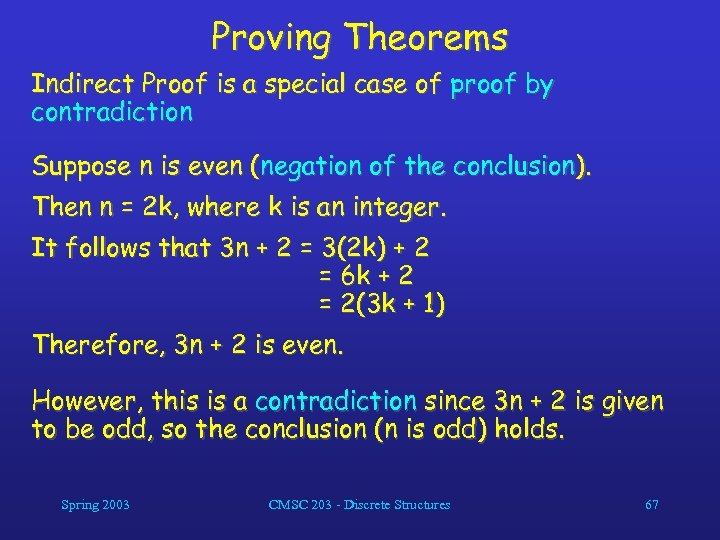Proving Theorems Indirect Proof is a special case of proof by contradiction Suppose n is even (negation of the conclusion). Then n = 2 k, where k is an integer. It follows that 3 n + 2 = 3(2 k) + 2 = 6 k + 2 = 2(3 k + 1) Therefore, 3 n + 2 is even. However, this is a contradiction since 3 n + 2 is given to be odd, so the conclusion (n is odd) holds. Spring 2003 CMSC 203 - Discrete Structures 67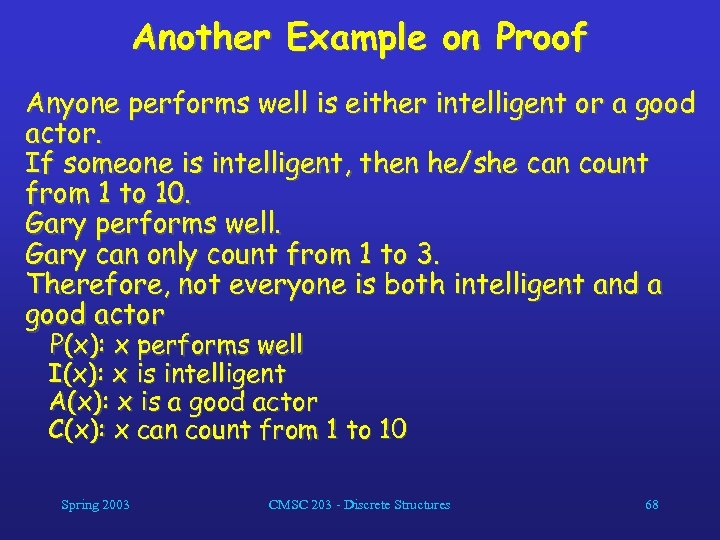Another Example on Proof Anyone performs well is either intelligent or a good actor. If someone is intelligent, then he/she can count from 1 to 10. Gary performs well. Gary can only count from 1 to 3. Therefore, not everyone is both intelligent and a good actor P(x): x performs well I(x): x is intelligent A(x): x is a good actor C(x): x can count from 1 to 10 Spring 2003 CMSC 203 - Discrete Structures 68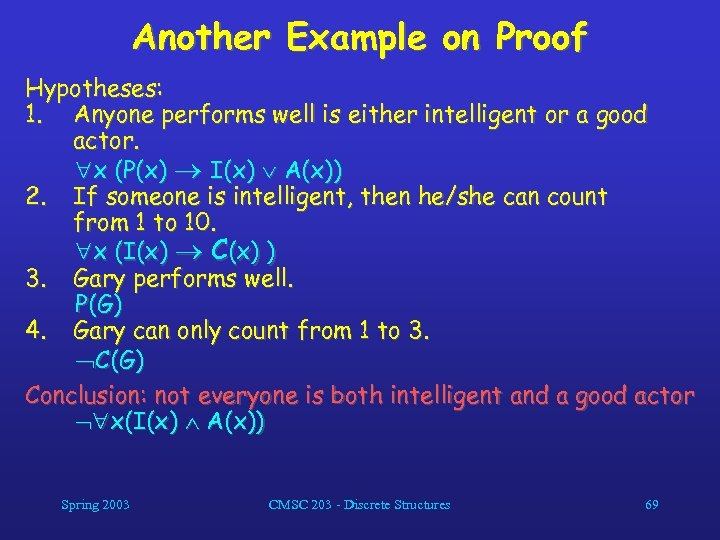Another Example on Proof Hypotheses: 1. Anyone performs well is either intelligent or a good actor. x (P(x) I(x) A(x)) 2. If someone is intelligent, then he/she can count from 1 to 10. x (I(x) C(x) ) 3. Gary performs well. P(G) 4. Gary can only count from 1 to 3. C(G) Conclusion: not everyone is both intelligent and a good actor x(I(x) A(x)) Spring 2003 CMSC 203 - Discrete Structures 69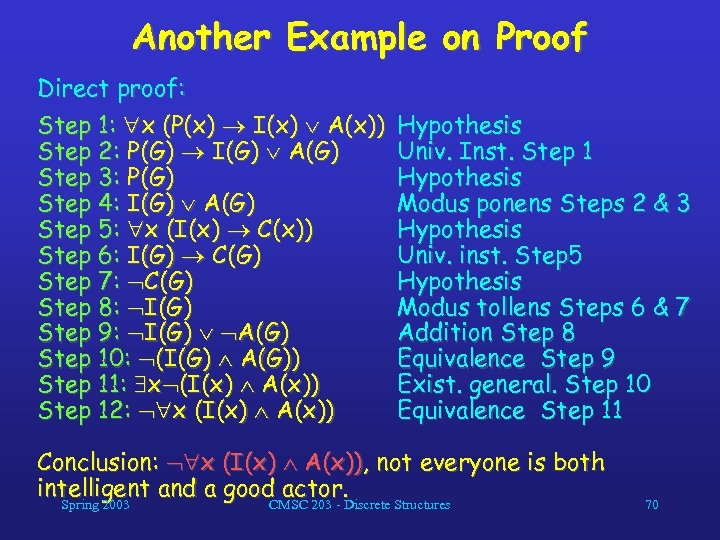Another Example on Proof Direct proof: Step 1: x (P(x) I(x) A(x)) Step 2: P(G) I(G) A(G) Step 3: P(G) Step 4: I(G) A(G) Step 5: x (I(x) C(x)) Step 6: I(G) C(G) Step 7: C(G) Step 8: I(G) Step 9: I(G) A(G) Step 10: (I(G) A(G)) Step 11: x (I(x) A(x)) Step 12: x (I(x) A(x)) Hypothesis Univ. Inst. Step 1 Hypothesis Modus ponens Steps 2 & 3 Hypothesis Univ. inst. Step 5 Hypothesis Modus tollens Steps 6 & 7 Addition Step 8 Equivalence Step 9 Exist. general. Step 10 Equivalence Step 11 Conclusion: x (I(x) A(x)), not everyone is both intelligent and a good actor. Spring 2003 CMSC 203 - Discrete Structures 70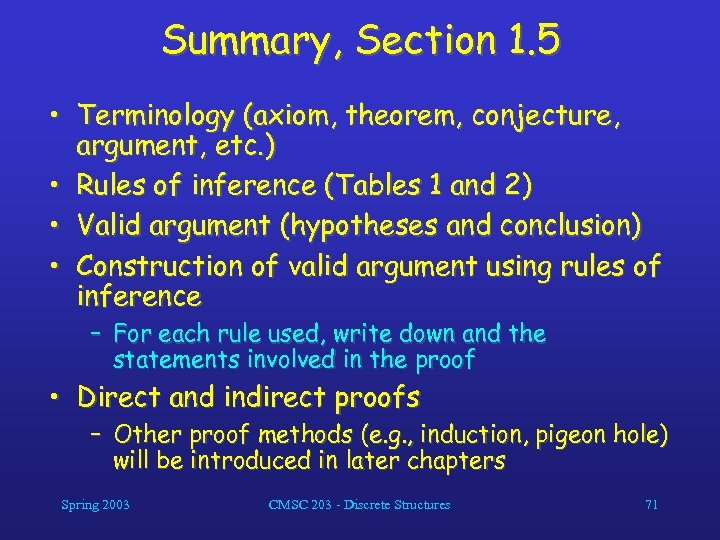Summary, Section 1. 5 • Terminology (axiom, theorem, conjecture, argument, etc. ) • Rules of inference (Tables 1 and 2) • Valid argument (hypotheses and conclusion) • Construction of valid argument using rules of inference – For each rule used, write down and the statements involved in the proof • Direct and indirect proofs – Other proof methods (e. g. , induction, pigeon hole) will be introduced in later chapters Spring 2003 CMSC 203 - Discrete Structures 71